# JP6147273B2 - Image registration method and system robust against noise - Google Patents

## Info

Publication number
JP6147273B2
JP6147273B2 JP2014547648A JP2014547648A JP6147273B2 JP 6147273 B2 JP6147273 B2 JP 6147273B2 JP 2014547648 A JP2014547648 A JP 2014547648A JP 2014547648 A JP2014547648 A JP 2014547648A JP 6147273 B2 JP6147273 B2 JP 6147273B2
Authority
JP
Japan
Prior art keywords
image
distorted
θ
module
reference image
Prior art date
Legal status (The legal status is an assumption and is not a legal conclusion. Google has not performed a legal analysis and makes no representation as to the accuracy of the status listed.)
Active
Application number
JP2014547648A
Other languages
Japanese (ja)
Other versions
JP2015503778A (en
Inventor
チェン，グァンイ
クーロン，ステファン
Original Assignee
エコール ドゥ テクノロジー スペリウールＥｃｏｌｅ Ｄｅ Ｔｅｃｈｎｏｌｏｇｉｅ Ｓｕｐｅｒｉｅｕｒｅ
エコール ドゥ テクノロジー スペリウールＥｃｏｌｅ Ｄｅ Ｔｅｃｈｎｏｌｏｇｉｅ Ｓｕｐｅｒｉｅｕｒｅ
Priority date (The priority date is an assumption and is not a legal conclusion. Google has not performed a legal analysis and makes no representation as to the accuracy of the date listed.)
Filing date
Publication date
Priority to US201161580200P priority Critical
Priority to US61/580,200 priority
Priority to US201261603347P priority
Priority to US61/603,347 priority
Application filed by エコール ドゥ テクノロジー スペリウールＥｃｏｌｅ Ｄｅ Ｔｅｃｈｎｏｌｏｇｉｅ Ｓｕｐｅｒｉｅｕｒｅ, エコール ドゥ テクノロジー スペリウールＥｃｏｌｅ Ｄｅ Ｔｅｃｈｎｏｌｏｇｉｅ Ｓｕｐｅｒｉｅｕｒｅ filed Critical エコール ドゥ テクノロジー スペリウールＥｃｏｌｅ Ｄｅ Ｔｅｃｈｎｏｌｏｇｉｅ Ｓｕｐｅｒｉｅｕｒｅ
Priority to PCT/CA2012/001195 priority patent/WO2013091088A1/en
Publication of JP2015503778A publication Critical patent/JP2015503778A/en
Application granted granted Critical
Publication of JP6147273B2 publication Critical patent/JP6147273B2/en
Application status is Active legal-status Critical
Anticipated expiration legal-status Critical

## Images

•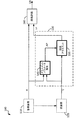••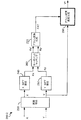•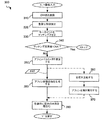•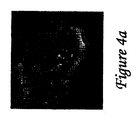•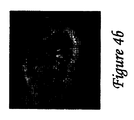•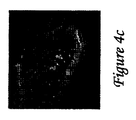•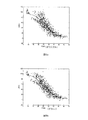•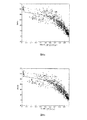•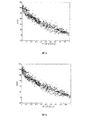•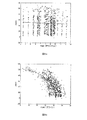•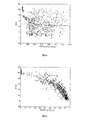•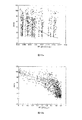•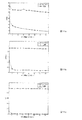•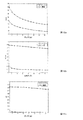•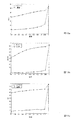•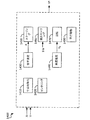•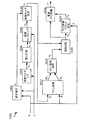•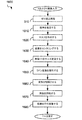•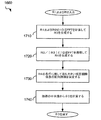•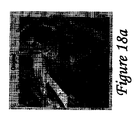•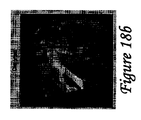•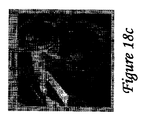•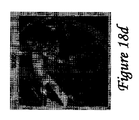•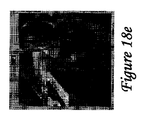•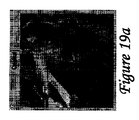•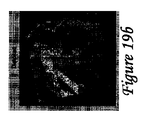•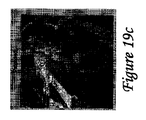•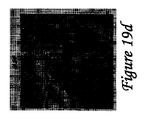••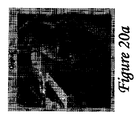•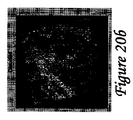•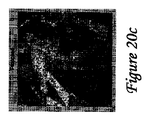••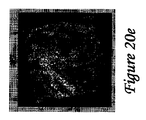•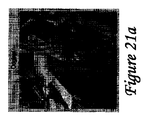•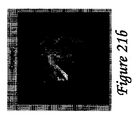•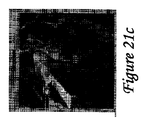•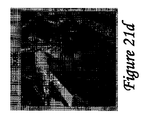•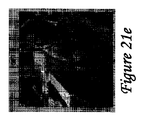•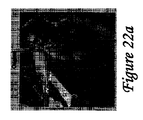•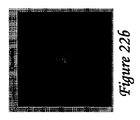•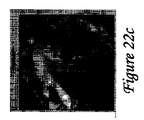•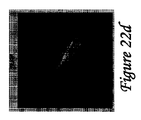•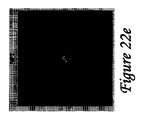•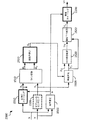•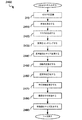•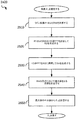•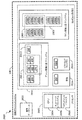## Classifications

• GPHYSICS
• G06COMPUTING; CALCULATING; COUNTING
• G06TIMAGE DATA PROCESSING OR GENERATION, IN GENERAL
• G06T3/00Geometric image transformation in the plane of the image
• G06T3/0068Geometric image transformation in the plane of the image for image registration, e.g. elastic snapping
• GPHYSICS
• G06COMPUTING; CALCULATING; COUNTING
• G06TIMAGE DATA PROCESSING OR GENERATION, IN GENERAL
• G06T7/00Image analysis
• G06T7/30Determination of transform parameters for the alignment of images, i.e. image registration
• G06T7/37Determination of transform parameters for the alignment of images, i.e. image registration using transform domain methods

## Description

The present invention relates generally to digital image processing, and more particularly to processing an image in combination with a reference image.

The present invention relates to image registration, including translation, rotation, and estimation of scaling parameters between a reference image and a distorted image. In particular, the present invention relates to image registration systems and methods that are robust against noise.

The present invention relates to a method and system for visual quality assessment of images (visual quality assessment), in particular for visual quality assessment of images, which is robust against affine transformations.

Image registration plays an important role in applications such as image quality evaluation, medical imaging, and automatic target recognition. Examples of existing image registration methods include the following. A. Averbuch, R.A. R. Coifman, D.C. L. Donoho, M.M. Israeli, Y. et al. Shkolnsky, and I.I. Sedelnikov's paper “A Framework for Discrete Integral Transformations II-The 2D Discrete Radon Transform”, SIAM Journal on Scientific Computation, Vol. 3, No. 80, No. 3, No. Using large translation, rotation, and scaling. G. Wolberg and S.W. Zokai's paper “Robust Image Registration Using Log-Polar Transform”, IEEE International Conference on Image Processing (ICIP) Proceedings, page 493-496, 2000 Worked on. G. Varghese and Z.M. Wang's papers “Video denoising based on a spatial temporal Gaussian scale mixture model, Volume 10 and IEEE Transac- tions on Circuits 10 and 20”. Global spatial displacement was estimated. B. S. Reddy and B.W. N. Article by Chatterji “An FFT-based technique for translation, rotation and scale-invariant image registration”, IEEE Transactions on Image Processing, Vol. 6, No. 6, No. 6, No. 126, No. 6, No. 126 And presents an FFT-based technique for scale-invariant image registration. K. Jafari-Khouzani and H.J. The paper by Soltian-Zadeh, “Radon transform orientation estimation for rotation invariant texture analysis, vol. 100, IEEE Transactions on Pattern Analysis, 100th gen. The radon transform is applied to the direction estimation. E. De Castro and C.I. Morandi's paper “Registration of translated and rotated imaged using finet Fourier transforms”, IEEE Transactions on Pattern Analysis and Machine Intelligence, Inc. PAMI-95, pages 700-703, 1987, developed an image registration method using a finite Fourier transform for translated and rotated images, which is not successful in the presence of scale changes. W. Wei, S .; Wang, X. et al. Zhang and Z. Article by Tang "Estimation of image rotation angle using interpolation-related spectral signatures with application to blind detection of image forgery", IEEE Transactions on Information Forensics and Security, Vol. 5, No. 3, No. 507-517 page, 2010 The image rotation angle was estimated using the spectral features related to the interpolation by the application of blind detection of image forgery. D. G. Low's paper “Distinctive Image Features from Scale-Invariant Keypoints”, International Journal of Computer Vision, Vol. 60, No. 2, pp. 91-110. A characteristic image characterization method for was presented. And H. Bay, A.M. Ess, T.E. Tuytelalas and L.C. Van Goul's paper "SURF: Speeded Up Robust Features", Computer Vision and Image Understanding (CVIU), Vol. 110, No. 3, pp. 346-359, 2008, SURF (Speeded Up Robus Development) , SURF claims to be faster than SIFT.

In assessing the quality of a complete reference image, a comparison of a reference image and a distorted image is often used to obtain a visual quality metric (visual quality metric). The metric is calculated by comparing two images. There are many ways to generate such quality metrics. For example: A simple peak signal to noise ratio (PSNR) measurement method; Wang, A.M. C. Bovik, H.M. R. Sheikh and E.I. P. Simoncelli's “Image quality assessment: From error visibility to structural similarity”, IEEE Transactions on Image Processing, Vol. 13, No. 4, pp. 600-612. H. R. Sheikh and A.K. C. Visual Information Fidelity (VIF) index presented in Bovik's “Image information and visual quality”, IEEE Transactions on Image Processing, Vol. 15, No. 2, pages 430-444. In addition, Rezadehde and Coulombe are “Novell Discrete Wavelet Transform for Full Reference Image Quality Quality Assessment”, Signal, Image and Video Processing for the Standard Image, 11th Month, 20th Annual Review A transformation framework is presented, which was published by Proceeda Engineering (Elsevering) as "A novel discrete domain error-based image quality metric with enhanced perceptual performance". Qian and Chen are "Four reduced-reference metrics for measuring hyperspectral images after spatial resolution enhancement", ISPRS International Archives of the Photogrammetry, Remote Sensing and Spatial Information Sciences, Vienna, Austria, the first 204-208 pages, July 2010 5 On the 7th, four metrics with reduced references were developed to measure the visual quality of hyperspectral images after increasing spatial resolution.

However, it is a concise but fast and accurate method for image realignment with reference images, avoiding or reducing the disadvantages of the prior art and improving the objective measurement of the visual quality of the image There is still a need in the industry for the development of methods that can be made to provide image registration that is robust against noise.

A. Averbuch, R.A. R. Coifman, D.C. L. Donoho, M.M. Israeli, Y. et al. Shkolnsky, and I.I. Sedelnikov, "A Framework for Discrete Integral Transformations II-The 2D Discrete Radon Transform", SIAM Journal on Scientific Computation, Vol. 3, Vol. 3, No. 3, No. 80 G. Wolberg and S.W. Zokai "Robust Image Registration Usage Log-Polar Transform", IEEE International Conference on Image Processing (ICIP), 493-496, 2000 G. Varghese and Z.M. Wang "Video denoising based on a spatial temporal Gaussian scale mixture model", IEEE Transactions on Circuits and Systems for Video 10th, 20th, 20th, 20th. B. S. Reddy and B.W. N. Chatterji "An FFT-based technique for translation, rotation and scale-invariant image registration", IEEE Transactions on Image Processing, Vol. 5, No. 6, No. 6, No. 6, No. 6, No. 6, No. 6, No. K. Jafari-Khouzani and H.J. Solantian-Zadeh, "Radon transform orientation estimation for rotation invariant texture analysis," vol. 4, IEEE Transactions on Pattern Analysis, 100, Chapter 6 E. De Castro and C.I. Morandi, "Registration of translated and rotated imaged using finet Fourier transforms," IEEE Transactions on Pattern Analysis and Machine Intelligence, Inc. PAMI-95, pages 700-703, 1987 W. Wei, S .; Wang, X. et al. Zhang and Z. Tang `` Estimation of image rotation angle using interpolation-related spectral signature with application of the 5th s of the 5th sine, the 5th s, the 5th s D. G. Low "Distinctive Image Features from Scale-Invariant Keypoints", International Journal of Computer Vision, Vol. 60, No. 2, pp. 91-110, 2004. H. Bay, A.M. Ess, T.E. Tuytelalas and L.C. Van Goul's paper "SURF: Speeded Up Robust Features", Computer Vision and Image Understanding (CVIU), Vol. 110, No. 3, pp. 346-359, 2008 Z. Wang, A.M. C. Bovik, H.M. R. Sheikh and E.I. P. Simoncelli, "Image quality assessment: From error visibility to structural similarity", IEEE Transactions on Image Processing, Vol. 13, No. 4, pages 600-612. H. R. Sheikh and A.K. C. Bovik "Image information and visual quality", IEEE Transactions on Image Processing, Vol. 15, No. 2, pages 430-444, 2006 Rezaadeh and Coulomb, "Novel discrete wavelet transform form full reference image quality assessment", Signal, Image and Video Processing, pages 1-15, 2011. Qian and Chen "Four reduced-reference metrics for measuring hyperspectral images after spatial resolution enhancement", ISPRS International Archives of the Photogrammetry, Remote Sensing and Spatial Information Sciences, Vienna, Austria, the first 204-208 pages, July 2010 5-7 Day LIVE image quality evaluation database release 2 R. Sheikh, Z. et al. Wang, L.W. Cormac and A.M. C. Bovik, http: // live. ce. utexas. edu / research / quality Z. Wang and Q.W. Li "Information content weighing for perceptual image quality assessment", IEEE Transactions on Image Processing, Vol. 20, No. 5, pp. 1185-1198, 2011 M.M. P, Sampat, Z.M. Wang, S.W. Gupta, A.M. C. Bovik and M.M. K. Sampat et al. "Complex wavelet structure similarity: A new image similarity index", IEEE Transactions on Image Processing, Vol. 18, No. 11, pp. 2385-2401, 2009 D. Nicolic, R.A. C. Muresan, W.M. Feng and W.W. Singer "Scaled correlation analysis: a better way to compute a cross-corregram", European Journal of Neuroscience, page 1-21, 2012 V. Sobolev "Convolution of functions", Michael Hazewinkel, Encyclopedia of Mathematicas, Springer, ISBN 977-1-55608-010-4 Y. Pan, K.M. Li and M.M. Hamdi "An improved constant-time algorithm for computing the 4th and 4th H, Transforms on H 4th, S and 4th H, Trans Transforms on the 4th, 29th and 29th, and the Transforms on the 4th. Page, 1999

An object of the present invention is to provide an image registration method for processing a first image Y into a registration image Y * aligned with / aligned with a second image X, and Is to provide a system.

According to one aspect of the invention, an image registration method for processing a first image Y into a registration image Y * aligned with a second image X, the method comprising:
(A) determining a scale factor “a” between the reference image X and the distorted image Y;
(B) resizing the distorted image Y at the magnification “a”, thereby generating a normalized distorted image Y2;
(C) determining a rotation angle “θ 0 ” between the reference image X and the normalized strain image Y2;
(D) rotating the normalized strain image Y2 by a rotation angle “−θ 0 ”, thereby obtaining a rotated strain image Y3;
(E) obtaining a registration image Y * by translating the rotated strain image Y3 so as to be aligned with the reference image X in a horizontal direction and a vertical direction, and an image registration method comprising: Provided.
In the above method, the step (a) includes the step of determining the magnification “a” by calculating a ratio of a sum of pixel values of the reference image X and a sum of pixel values of the distorted image Y.

In the step (a), the smaller one of the reference image X and the distorted image Y is padded with zero-valued pixels, and the horizontal and vertical dimensions of the reference image X and the distorted image Y (dimension). ) Are further included.

The step (b) centers the distorted image Y, thereby forming a centered distorted image Y1, resizes the centered distorted image Y1 at the magnification “a”, and normalizes the distorted image Y1. The method further includes generating an image Y2.

The step (c) forms Radon transforms R1 and R2 of the reference image X and the normalized distorted image Y2, respectively, calculates a cross-correlation between the rows of the Radon transforms R1 and R2, and calculates the rotation angle “ determining θ 0 ”.

The step of calculating the cross correlation includes the following steps.
(I) calculating a set of circumferential cross-correlations between each row of the Radon transform R1 and each row of the Radon transform R2, wherein each circumferential cross-correlation is calculated for each row; Defining a rotational offset angle “θ” between.
(Ii) selecting a circumferential cross-correlation having the highest value for each row;
(Iii) Determine a rotational offset “θ” defined by each selected circumferential cross-correlation for each row, and determine the rotational angle “θ 0 ” as the median of the determined rotational offset “θ” Setting to be equal to.

The step of calculating the cross correlation further includes the following steps.
(Iv) calculating a one-dimensional (1D) fast Fourier transform (FFT) of the Radon transform R1 and the Radon transform R2.
(V) combining the 1D FFT of the Radon transform R1 and the Radon transform R2 into an intermediate result R3.
(Vi) generating a second intermediate result R4 including the circumferential cross-correlation, wherein the second intermediate result R4 is based on elements of the intermediate result R3.
(Vii) selecting a circumferential cross-correlation having the highest value in each row of the intermediate result R3.
(Viii) determining the corresponding rotational offset “θ” defined in each selected circumferential cross-correlation and making the rotational angle “θ 0 ” equal to the median value of the determined rotational offset “θ” Step to set.

The combining step includes forming a component-by-component product of the 1D FFT of the Radon transform R1 and the “conjugate” function of the 1D FFT of the Radon transform R2.

In the above method, the step (vi) further includes a step of determining a maximum value corresponding to each angle “θ” for each element row in the second intermediate result R4. The method further includes taking a median value of the maximum values, the median value corresponding to a rotation angle “θ 0 ”.

The method performs a visual quality assessment of the preprocessed image Y * , eg, visual quality assessment, peak signal noise ratio (PSNR) determination, structural similarity (SSIM) index calculation, visual information Performing any one of the fidelity (VIF) index calculations.

According to another aspect of the present invention, there is an image registration method for processing a first image into a registration image Y * aligned with a second image, the method comprising:
(A) determining a magnification “a” between the first image and the second image;
(B) Resizing the first image at the magnification “a” to generate a resized version of the first image, thereby reducing the size of the first image and the second image Equalizing steps;
(C) determining a rotation angle “θ 0 ” between the resized version of the first image and the second image using a Radon transform;
(D) Rotate the resized version of the first image by a rotation angle “−θ 0 ”, translate it to align with the second image in the horizontal and vertical directions, and then register Outputting as image Y * , an image registration method is provided.

According to yet another aspect of the present invention, a system for processing a distorted image Y into a registration image Y * by aligning with a reference image X, the system comprising:
A processor;
A memory device having stored thereon computer readable instructions for execution by the processor,
A magnification estimation module that determines the magnification "a";
An image resizing module that resizes the distorted image Y to a resized distorted image Y2 using the magnification "a";
A rotation angle determination unit for estimating the rotation angle “θ 0 ”;
An image rotation module that forms a corrected image Y3 by rotating the resized distorted image Y2 by a rotation angle “−θ 0 ”;
An image translation module is provided that translates the corrected image Y3 to generate the registration image Y * .

In the above system, the rotation angle determination unit includes:
A Radon transform module for generating Radon transforms R1 and R2 of the resized strain image Y2 and the reference image X, respectively;
And a Radon transform correlation module for estimating a rotation angle “θ 0 ” from the Radon transforms R2 and R1.
The system further includes an image evaluation module processing module for performing visual quality evaluation of the registration image Y * .

In the above system, the image evaluation module processing module performs visual quality evaluation by any one of the following.
Determine the peak signal-to-noise ratio (PSNR).
Calculate structural similarity (SSIM) index.
Calculate visual information fidelity (VIF) index.

The system
An image centering module for centering the distorted image Y to generate a centered image Y1;
The image resizing module is configured to perform resizing of the centered image Y1 to the resized distorted image Y2 using the magnification “a”.

The system
An image masking module for extracting a masked image Y0 from the distorted image Y; and a centroid calculating module for determining centering parameters “cr” and “cc” from the masked image Y0;
The image centering module is configured to generate the centered image Y1 from the distorted image Y using the centering parameters “cr” and “cc”.

The system
A translation estimation module for determining an offset vector “TV” between the corrected image Y3 and the reference image X;
The image translation module is configured to translate the corrected image Y3 using the offset vector “TV” to generate the registration image Y * .

A system and method of yet another embodiment of the invention is described below.

According to yet another aspect of the present invention, there is provided an image registration method for processing a distorted image Y into a registration image Y * aligned with a reference image X,
(A) determining a magnification “a” between the reference image X and the distorted image Y;
(B) resizing the reference image X by the inverse of the magnification “a”, thereby generating a resized reference image X 2;
(C) determining a rotation angle “θ 0 ” between the resized reference image X2 and the distorted image Y;
(D) determining a rotated strain image Y3 by rotating the strain image Y by a rotation angle “−θ 0 ”;
(E) resizing the rotated distorted image Y3 at the magnification “a”, thereby obtaining the registration image Y * , an image registration method is provided.

In the above method, the step (a) includes a step of determining a magnification “a” by calculating a ratio of a sum of pixel values of the reference image X and a sum of pixel values of the distorted image Y.

The step (a) includes the step of embedding a smaller one of the reference image X and the distorted image Y with pixels having a zero value, and equalizing the horizontal and vertical dimensions of the reference image X and the distorted image Y. In addition.

The step (c) includes a step of centering the distorted image Y to generate a centered distorted image Y1, and a rotation angle “θ between the resized reference image X2 and the centered distorted image Y1. Further comprising the step of making a “ 0 ” determination.

The step (c) calculates the cross-correlation between the steps of forming the Radon transforms R1 and R2 of the resized reference image X2 and the distorted image Y, respectively, and the rows of the Radon transforms R1 and R2, and Determining a rotation angle “θ 0 ”.

The step (c) calculates a cross-correlation between the steps of forming Radon transforms R1 and R2 of the resized reference image X2 and the centered distortion image Y1, respectively, and the rows of the Radon transforms R1 and R2. And determining the rotation angle “θ 0 ”.

Calculating the cross-correlation comprises:
(I) calculating a set of circumferential cross-correlations between each row of the Radon transform R1 and each row of the Radon transform R2, wherein each circumferential cross-correlation is calculated between the rows; Defining a rotational offset angle “θ”; and
(Ii) selecting a circumferential cross-correlation having the highest value for each row;
(Iii) Determine the rotational offset “θ” defined by each selected circumferential cross-correlation for each row, and set the rotational angle “θ 0 ” to the median value of the determined rotational offset “θ”. And setting the same.

Calculating the cross-correlation comprises:
(Iv) calculating a one-dimensional (1D) fast Fourier transform (FFT) of the Radon transform R1 and the Radon transform R2;
(V) combining the 1D FFT of the Radon transform R1 and the Radon transform R2 into an intermediate result R3;
(Vi) generating a second intermediate result R4 including the circumferential cross-correlation, wherein the second intermediate result R4 is based on elements of the intermediate result R3;
(Vii) selecting a circumferential cross-correlation having the highest value in each row of the intermediate result R3;
(Viii) determining the corresponding rotational offset “θ” defined in each selected circumferential cross-correlation and setting the rotational angle “θ 0 ” equal to the median value of the determined rotational offset “θ” Further comprising the step of:

The combining step (v) includes forming a product for each component of the 1D FFT of the Radon transform R1 and the “conjugate” function of the 1D FFT of the Radon transform R2.

The step (vi) includes a step of determining a maximum value corresponding to each angle “θ” for each row in the second intermediate result R4.

The method further includes selecting the median value of the maximum value and the rotation angle “θ 0 ”, wherein the rotation angle “θ 0 ” corresponds to the angle referenced by the cross-correlation indicated by the median value. To do.

The method further includes performing a visual quality assessment of the registration image Y * .

13. The method of claim 12, wherein visual quality assessment is performed by either peak signal noise ratio (PSNR) determination, structural similarity (SSIM) index calculation, or visual information fidelity (VIF) index calculation.

According to another aspect of the invention, an image registration method for processing a first image into a registration image Y * aligned with a second image, comprising:
(A) determining a magnification “a” between the first image and the second image;
(B) generating a resized version of each of the images by resizing one of the images at the magnification “a” to equalize the sizes of the first image and the second image;
(C) determining a rotation angle “θ 0 ” of the first image and the second image or their corresponding resized version using a Radon transform;
(D) Rotate the first image by a rotation angle “−θ 0 ” and translate it so that it is aligned horizontally and vertically with the second image of the resized version. , After resizing at the magnification “a”, and outputting as the registration image Y * .

According to yet another aspect of the present invention, a system for processing a distorted image Y into a registration image Y * by aligning it with a reference image X, comprising:
A processor;
A computer readable storage medium having stored thereon computer readable instructions for execution by the processor,
A magnification estimation module for determining a magnification “a” between the reference image X and the distorted image Y;
A rotation angle determination unit that estimates a rotation angle “θ 0 ” between the reference image X and the distorted image Y;
An image rotation module that forms a rotated distortion image Y3 by rotating the distortion image Y by a rotation angle “−θ 0 ”;
A system is provided for forming an image scaling module that resizes the rotated distorted image Y3 to generate a registration image Y * .

The system further includes an optional centering module for generating a centered image Y1, wherein the rotation angle determination unit is configured to rotate the rotation angle “θ 0 between the reference image X and the centered image Y1. Is estimated.

The system further includes an image pre-scaling module that scales the reference image X by a reciprocal of the magnification “a” into a resized reference image X2, and the rotation angle determination unit includes the resized reference image The rotation angle “θ 0 ” between X2 and the centered image Y1 is estimated.

The system further includes a translation estimation module that determines an offset vector “TV” between the corrected image Y3 and the reference image X, the image translation module including the rotated distortion image Y3 as the The registration image Y * is generated by translation using the offset vector “TV”.

The system further includes an image evaluation module processing module for performing visual quality evaluation of the registration image Y * .

The image evaluation module is configured to perform visual quality evaluation by any of the following: Determine the peak signal-to-noise ratio (PSNR). Calculate structural similarity (SSIM) index. Calculate visual information fidelity (VIF) index.

In this way, improved image registration methods and systems are provided.

Embodiments of the present invention will be more fully understood from the following detailed description of preferred embodiments that should be read with reference to the accompanying drawings. These drawings should not be construed as limiting the present application, but are intended to be exemplary only.

1 is a schematic block diagram of an image alignment system 100. FIG. FIG. 6 is a block diagram of affine transformation based on the image alignment system 200.1. FIG. 6 is a block diagram of an alternative affine transformation based on the image alignment system 200.2 that is the second embodiment of the present invention. 4 is a flowchart of affine transformation based on the realignment method 300 according to the first embodiment of the present invention. 4 is an image of the result of affine transformation based on the realignment method 300 of FIG. 4 is an image of the result of affine transformation based on the realignment method 300 of FIG. 4 is an image of the result of affine transformation based on the realignment method 300 of FIG. 5 to 13 are image results displaying the experimental results obtained by the realignment method 300 of FIG. 3 in more detail. FIG. 5a is a scatter plot of PSNR scores (horizontal axis) and DMOS (vertical axis) for “original” measurements. FIG. 6 is a corresponding scatter plot for measurement after affine preprocessing of an image. FIG. 6 is a scatter plot of MSSIM scores (horizontal axis) and DMOS (vertical axis) for “original” measurements. FIG. 6 is a corresponding scatter plot for measurement after affine preprocessing of an image. It is a scatter diagram of a VIF score (horizontal axis) and a DMOS (vertical axis) regarding the measurement of “original”. FIG. 6 is a corresponding scatter plot for measurement after affine preprocessing of an image. FIG. 7 is a scatter plot of PSNR scores (horizontal axis) and DMOS (vertical axis) for all distorted images in the LIVE image database with additional affine transformations for “original” measurements. FIG. 6 is a corresponding scatter plot for measurement after affine preprocessing of an image. FIG. 6 is a scatter plot of MSSIM scores (horizontal axis) and DMOS (vertical axis) for all distorted images in the LIVE image database with additional affine transformations for “original” measurements. FIG. 6 is a corresponding scatter plot for measurement after affine preprocessing of an image. FIG. 6 is a scatter plot of VIF scores (horizontal axis) and DMOS (vertical axis) for all distorted images in the LIVE image database with additional affine transformations for “original” measurements. FIG. 6 is a corresponding scatter plot for measurement after affine preprocessing of an image. FIG. 6 is a graph of results of experiments that distort selected images in a LIVE database by translation with respect to PSNR metrics. FIG. 6 is a graph of results of experiments that distort selected images in a LIVE database by translation with respect to MSSIM metrics. FIG. 6 is a graph of experimental results that distort selected images in a LIVE database by translation with respect to a VIF metric. FIG. 5 is a graph of experimental results that distort selected images in a LIVE database with rotations from 1 to 15 degrees with respect to PSNR metrics. FIG. 6 is a graph of experimental results that distort selected images in a LIVE database with rotations from 1 to 15 degrees with respect to MSSIM metrics. FIG. 6 is a graph of experimental results that distort selected images in a LIVE database with rotations from 1 to 15 degrees with respect to a VIF metric. FIG. 6 is a graph of experimental results that distort selected images in a LIVE database with scaling from 0.5 to 1.0 magnification with respect to the PSNR metric. FIG. 6 is a graph of experimental results that distort selected images in a LIVE database with scaling from 0.5 to 1.0 magnification with respect to MSSIM metrics. FIG. 5 is a graph of experimental results that distort selected images in a LIVE database with scaling from 0.5 to 1.0 magnification with respect to the VIF metric. FIG. 1B shows a block diagram 1400 for an alignment system based on Radon transform. It is a block diagram of the image alignment system 1500 based on 1st Radon transform which is the 3rd Embodiment of this invention. FIG. 16 is a diagram of a method 1600 based on a first Radon transform for the system of FIG. FIG. 17 is a development view of step 1660 “correlation transformation” in FIG. 16. The result of the experiment which shows operation | movement of the 4th Embodiment of this invention described in FIG. 23 is shown. Fig. 4 shows an original image, a distorted version thereof, a corresponding distorted image registered by the method of the third embodiment of the invention and the method of the prior art. The result of the experiment which shows operation | movement of the 4th Embodiment of this invention described in FIG. 23 is shown. Fig. 4 shows an original image, a distorted version thereof, a corresponding distorted image registered by the method of the third embodiment of the invention and the method of the prior art. The result of the experiment which shows operation | movement of the 4th Embodiment of this invention described in FIG. 23 is shown. Fig. 4 shows an original image, a distorted version thereof, a corresponding distorted image registered by the method of the third embodiment of the invention and the method of the prior art. The result of the experiment which shows operation | movement of the 4th Embodiment of this invention described in FIG. 23 is shown. Fig. 4 shows an original image, a distorted version thereof, a corresponding distorted image registered by the method of the third embodiment of the invention and the method of the prior art. The result of the experiment which shows operation | movement of the 4th Embodiment of this invention described in FIG. 23 is shown. Fig. 4 shows an original image, a distorted version thereof, a corresponding distorted image registered by the method of the third embodiment of the invention and the method of the prior art. It is a block diagram of the image alignment system 2300 based on extended Radon transform which is the 4th Embodiment of this invention. FIG. 24 shows a method 2400 based on a second Radon transform for the system of FIG. FIG. 25 is a development view of step 2450 “estimation of rotation angle” in FIG. 24. 2 is a composite block diagram 2600 of an embodiment of an image alignment system 100. FIG.

The present invention presents a system and method for preprocessing a distorted image that aligns the distorted image with a reference image prior to calculating a quality metric. The process of aligning the reference image with the distorted image (or the process of aligning the reference image with the distorted image, the process of aligning the reference image with the distorted image) is “registering” or “registration”. May also be called.

The full reference method of image quality evaluation of a distorted image is based on a special form comparison between the distorted image and the reference image. If the distortion involves a dimensional difference between the two images, a change in geometric properties such as horizontal and / or vertical displacement or rotation of the distortion image within its envelope, or other deformations, Existing full reference quality assessment methods do not generate quality metrics (metrics) that can accommodate visual assessments.

Other applications of the image registration method presented in the embodiments of the present invention appear (almost) the same in visual observation, but differ in that they are scaled, rotated, or one image is noisy, etc. Automatic comparison of two images.

Therefore, image registration is applied to remote sensing (map update) and computer vision.

Medical image registration (for example, image data of the same patient taken at different times, eg, change detection or tumor monitoring) can be elastic (nonrigid) due to, for example, respiratory or anatomical changes. )) (Also known as) can be used to compensate for the deformation. Non-rigid registration of medical images is also useful for registering patient image data to anatomical atlases, such as the Talayrach atlas for neuroimaging.

A further use of image registration is to align images taken of the universe in astrophotography. Control points (automatically or manually entered) can be used to transform key features on one image to align with a second image.

Image registration is an essential part of panoramic image generation. The technology presented in the present invention is implemented and implemented in real time in embedded devices such as cameras and camera-equipped mobile phones.

FIG. 1 illustrates a reference image source 110 that provides a reference image X, a distorted image source 120 that provides a distorted image Y, an image preprocessing system 130 that includes an alignment parameter estimation process 140 and an image alignment process 150, and image evaluation. A schematic block diagram of an image alignment system 100 having a process 160 is shown.

Both the reference image X and the distorted image Y are input to the image preprocessing system 130. Here, the distorted image Y is preprocessed with reference to the base image X to generate a preprocessed image Y * , which is also called a registration image Y * .

In general, the image evaluation process 160 receives as input the reference image X and the preprocessed image Y * and generates an evaluation result Q0.

The distorted image Y may be traced back to the reference image X, and the distortion of the distorted image Y is a geometry characterized by compression and subsequent decompression of the image; image rotation, translation, or linear scaling. May be the result of either alone or in combination of the following: distorted image; loss of part of image due to cropping; addition of noise due to transfer over noisy channel.

The purpose of the alignment parameter estimation process 140 is to analyze the distorted image Y with reference to the reference image X and generate a set of alignment parameters AP that provide an estimate of the geometric features of the distortion.

The alignment parameter AP is used by an image alignment process 150 that transforms the distorted image Y into a preprocessed image Y * . The preprocessed image Y * may also be referred to as a registration image Y * . Using the alignment parameter AP, the image alignment process 150 compensates for some or all geometric distortion of the distorted image Y, but does not change the visual properties of the image itself. As a result, the distorted image Y is transformed into a registration image Y * by the image alignment process 150. That is, it is registered and aligned with the reference image X.

The image evaluation process 160 may be based on conventional visual quality measurements, in which case the evaluation result Q0 is a quality metric such as a structural similarity (SSIM) index or visual information fidelity (VIF) index ( Quality metric). In this case, the evaluation result Q0 is calculated for the preprocessed image Y * but is assumed as the true visual quality metric of the distorted image Y. This is because the preprocessing action only corrects the geometric distortion without changing the visual content of the image. For example, noise and compression effects remain.

In many other applications, the output Q0 of the image evaluation process 160 is simply a visual similarity between the distorted image Y and the reference image X, for example when both images are separate photographs of the same scene. It can be an indicator.

The first embodiment of the image preprocessing system 130 is based on the following.
-Extracting a set of keypoints from both the reference image X and the distorted image Y;
Matching the closest number of keypoint pairs selected from X and Y.
Solving for the parameters of the first affine transformation.
-Generating a second affine transformation that is the inverse of the first affine transformation;
Applying a second affine transformation to the distorted image Y, thereby generating a preprocessed image Y * .

Key points that characterize important regions in the image are: G. Low “Different Image Features from Scale-Invariant Keypoints”, International Journal of Computer Vision, Volume 60, No. 2, pages 91-110, scale change presented by IF T ing. Two specific embodiments of the alignment parameter estimation process 140 and corresponding embodiments of the image alignment process 150 are presented.

FIG. 2 a shows a block diagram of an image alignment system 200.1 based on an affine transformation, which is a first embodiment of image preprocessing system 130. The system 200.1 includes an affine transformation parameter estimation subsystem 210 that is an embodiment of the alignment parameter estimation process 140 of FIG. 1 and an affine image transformation module 220 that is an embodiment of the image alignment process 150 of FIG.

The affine transformation parameter estimation subsystem 210 includes a pixel embedding module 230, an X-SIFT extraction module 240, a Y-SIFT extraction module 250, a keypoint matching module 260, an affine transformation generation module 270, and a transformation inversion module 280.

In the pixel embedding module 230, if the two images X and Y are not the same with respect to the horizontal dimension “M” and the vertical dimension “N” measured in the pixel, the two images X and Y are changed. It is passed without being. If the images are not the same size, they are modified to be the same with respect to the horizontal and vertical dimensions by appropriately embedding one or both of the images with zero value pixels (for each dimension). Embed the image with the minimum resolution of its dimensions (direction)). Images having the same dimensions are referred to as an equalization reference image X ′ and an equalization distortion image Y ′. They are all M × N pixels in size.

For the sake of brevity, the equalization reference image X ′ and the equalization distortion image Y ′ are understood with the understanding that pixel embedding may not actually be necessary in any given case. Subsequently, they are simply referred to as a reference image X and a distorted image Y. For those familiar with the concept of image processing, if pixel embedding has been performed, the processing step (not shown in the description of the embodiment) is performed by deleting the row or column of pixels from the registration image Y * . It will be understood that this can be done to ensure that the dimensions of the image Y * match the dimensions of the (true) reference image X.

In the X-SIFT extraction module 240 and the Y-SIFT extraction module 250, the structure of scale invariant feature transformation (SIFT) is the same as that in D.S. G. Image as described by Low, "Distinctive Image Features from Scale-Invariant Keypoints", International Journal of Computer Vision, Volume 60, No. 2, pages 91-110, Y, X Each is extracted in the form of a set of key points Kx and Ky.

The keypoint set Kx and Ky is input to the keypoint matching module 260.

In the keypoint matching module 260, a predetermined number of keypoints is selected from the keypoint set “Kx” and “Ky” as matched keypoint subsets Kx ′ and Ky ′, which is the closest matching feature vector. Have. The selected keypoint subsets Kx ′ and Ky ′ are affine transformation generation modules to calculate the parameters of the forward affine transformation “FAT” that most closely approximates the geometric relationship between the reference image X and the distorted image Y. 270. The FAT is sent to the conversion inversion module 280 and inverted to generate an inverse affine conversion IAT. This represents a version of the first embodiment of the set of alignment parameters of FIG.

In the affine transformation parameter estimation subsystem 210, the inverse affine transformation IAT is applied to transform the distorted image Y into a registration image Y * .

FIG. 2 b is a block diagram of an image alignment system 200.2 based on an alternative affine transformation, which is a second embodiment of the image preprocessing system 130. The alternative affine transformation based image alignment system 200.2 of FIG. 2b except that the transform inversion module 280 and the affine image transformation module 220 are combined into a single, more efficient affine compensated image generation module 290. Thus providing the same functionality as the image alignment system 200.1 based on the affine transformation of FIG. 2a.

FIG. 3 is a flowchart of a realignment method 300 based on an affine transformation according to the first embodiment of the present invention, which includes the following steps.
310 “Zero Embedding Image”
320 “Important Feature Extraction”
330 “Match key points”
340 “Number of matching pixels <NK?”
350 “Estimate Affine Parameters”
360 “Invert Conversion”
370 “Perform affine transformation”
380 “Generate affine compensated image” and 390 “Embed blank pixels”

The reference image X is represented as x (i, j) (i = 1 to m, j = 1 to n), and the distorted image Y is represented by y (i, j) (i = 1 to m ′, j = 1 to n ′). ). The reference image X can be a distorted image Y that has undergone various distortions including compression, noise contamination, and possible affine transformations. It is not considered that the two images (X and Y) are completely different images.

In step 310 “zero-embedded image” executed in the image embedding module 230 of FIG. 2 a, the dimensions m and n of the reference image X are compared with the corresponding dimensions m ′ and n ′ of the distorted image Y.

If the reference image X and the distorted image Y do not have the same number of images, the dimensions of the equalized images X and Y are set to M and N. Here, M is the larger of m and m ', and N is the larger of n and n'. Either image is embedded by adding zero pixels around the original image in a small dimension, and then the equalized reference image X ′ or equivalent to replace the reference image X and the distorted image Y as the case may be A distorted image Y ′ can be generated. For the sake of brevity, the images will be referred to as the reference image X and the distorted image Y regardless of whether or not embedding has been performed.

In step 320 “Extract Key Feature” performed in the X-SIFT extraction module 240 and the Y-SIFT extraction module 250 of FIG. 2a, the scale-invariant feature transformation (SIFT) comprises the reference image X and In the distorted image Y, local (local) features are calculated to detect and describe, respectively. The detailed method is described by David Low, “Distinctive Image Features from Scale-Invariant Keypoints”, International Journal of Computer Vision, Vol. 60, No. 2, pages 91-110.

SIFT keypoints are extracted from both the reference image X and the distorted image Y. Following David Low's “SIFT” approach, a list of keypoints is obtained, where each keypoint is a local descriptor vector, ie a position in the image, and a visual representation of the content such as color, texture, etc. Contains a description of the feature. For the reference image X and the distorted image Y, a plurality of Kx and Ky keypoints (or sets thereof) are obtained by this method, respectively. Each keypoint is represented by a descriptor vector of 128 values, and may be associated with a position record containing four values: row index, column index, scale factor, and keypoint direction.

The keypoint descriptor is then normalized to represent a point in the N-dimensional Euclidean space. Here, N = 128, which corresponds to 128 values of each descriptor.

In step 330 “match keypoints” performed in the keypoint matching module 260 of FIG. 2a, the number NK of close matches between the keypoints of the reference image X and the distorted image Y is calculated. Is done. Here, the closeness of matching is determined by a distance value in a 128-dimensional space. For keypoint features, matching is only allowed if the distance is less than the distRatio times the second closest matching. The parameter distRatio may be selected within the range of 0.2 to 0.6. A value of 0.4 for distRatio is used in the following experiments.

For computational efficiency, it may be more efficient to calculate the dot product between unit vectors rather than the true Euclidean distance. Note that the angle ratio calculated by the unit product dot product acos is a good approximation of the Euclidean distance ratio for small angles. Therefore, matching is maintained only when the ratio of the vector angle of the nearest point to the vector angle of the second nearest neighbor point is smaller than distRatio.

In step 340 “number of matching pixels <NK?”, It is determined whether at least NK keypoint pairs matching in the previous step 330 have been found. The value of NK is preferably set to 4. If the number of matching pixel pairs found is less than 4 (Yes from step 340), it is impossible to perform affine preprocessing of the distorted image Y and the function must stop. Otherwise (No exit from step 340), execution continues to step 350.

In step 350 “estimate affine parameters” performed in the affine transformation generation module 260 of FIG. 2a, a global affine transformation between matching keypoint pairs may be determined using a linear least squares method.

Specifically, parameters in the affine transformation are determined as follows.

The affine transformation from the point [i k j k ] T of the reference image to the point [u k v k ] T of the distorted image is described as follows.
Here, k is an index of matching key points. The unknown variables are the conversion parameters a 1 , a 2 , a 3 , a 4 , t 1 and t 2 . Equation (1) is rewritten as:
Here, each set of matching keypoints contributes to the two rows of the leftmost matrix and the rightmost vector. The rightmost vector is thus [u 1 v 1 u 2 v 2 . . . u NK v NK ] T. This equation can be solved by the linear least squares method. Equation (2) is abbreviated as PX = Q. Therefore,
X = (P T P) −1 P T Q (3)
Get.

Thus, the transformation parameters are a 1 = X (1), a 2 = X (2), a 3 = X (3), a 4 = X (4), t 1 = X (5), and t 2 = X (6).

The output of step 350 “estimate affine parameters” is therefore the set of affine transformation parameters a1, a2, a3, a4, t1 and t2, derived as described above. It shows a forward affine transformation (FAT) between the reference image X and the distorted image Y. The distorted image can be manipulated geometrically to resemble the reference image X by applying FAT inversion to the distorted image. That is, it can be scaled, rotated, shifted (translated), and even sheared. Thereby, the distorted image Y is converted into a registration image Y * , for example, an affine compensated image y * (i * , j * ), which has the same resolution as the reference image X.

[i * j * ] T is calculated in two ways for an affine compensated image y * (i * , j * ) where i * ∈ [1, m] and j * ∈ [1, n]. Can do.

In step 360 “invert transform” performed in transform inversion module 260 of FIG. 2a, the inverse affine transformation is performed as follows.
Thereafter, step 370 “execute affine transformation” executed in the affine image transformation module 220 in FIG. 2A is performed. The preprocessed image is then
y * (i * , j * ) = y (u, v) (5)
As obtained.

However, since the calculated values i * and j * may not be integer values, the problem of finding the elements of y * (i * , j * ), the affine compensated image y * at integer positions, needs to be addressed. (Exists but is not explicitly stated in step 370).

In the preferred step 380 “Generate Affine Compensated Image” performed in the affine compensated image generation module 290 of FIG. 2b, the functions of steps 360 and 370 described above are combined to project the pixels directly into integer exponent positions. An affine compensation image y * (i * , j * ) is generated. The pixel value is calculated by bilinear interpolation as follows.

The following formula is established for all the pixels (i * , j * ) of the affine compensation image y * (i * , j * ).

Since the modified index value (u, v) T is a real value, bilinear interpolation can be used to calculate the value y * (i * , j * ).

If the index values u and v are in the range 1 ≦ u ≦ m and 1 ≦ v ≦ n, equation (7) below provides a simplified representation of the process of step 380.
here,
and
Are the ceiling function and floor function of real value u, respectively. In contrast, if u or v is outside these ranges, ie u <1 or m <u or v <1 or n <v, the corresponding pixel value is assigned to 0: y * (i * , J * ) = 0.

An exemplary embodiment of step 380, ie equations (6) and (7), is further illustrated in the following MATLAB code sample 1. Note: MATLAB (matrix laboratory) is a numerical computing environment and fourth generation programming language developed by Math-Works (http://www.mathworks.com). MATLAB sample 1

In step 390 “optionally fill blank pixels”, any blank pixels near the image boundary of the affine compensated image y * may be left blank, and optionally non-blank image pixels at the image boundary. It may be embedded according to the mirroring value.

For example, if you have the following image:

In this case, embedding of missing pixels (zero values) with mirroring values is performed by mirroring the non-blank pixel values at the boundary between the blank and non-blank values. This gives:

For the purposes of the evaluation of the first embodiment of the present invention, these pixels are left blank.

In short, the realignment method 300 based on affine transformation receives a reference image X and a distorted image Y as inputs and outputs an aligned registration image Y * using a SIFT technique combined with an affine transformation technique.

FIGS. 4a, 4b and 4c show the image results of the realignment method 300 based on the affine transformation of FIG. The original image used in place of the reference image X is shown in FIG. To illustrate the affine transformation-based realignment method 300, the affine transformation of the original image of FIG. 4a creates one distorted image for use as the distorted image Y, which is scaled, rotated, translated, and sheared. Presents a combination of distortion. The distorted image Y is shown in FIG. The distorted image Y of FIG. 4b was preprocessed by the realignment method 300 based on the affine transformation of FIG. 3 to generate a preprocessed image, ie, the registration image Y * shown in FIG. Visual inspection shows that the preprocessing method can perform excellent work in aligning the distorted image to the reference image.

To test the performance of the realignment method 300 based on affine transformation, R. Sheikh, Z. et al. Wang, L.W. Cormac and A.M. C. Published by Bovik, http: // live. ce. utexas. A number of experiments were performed using images from the LIVE image quality assessment database release 2 available on the Internet from edu / research / quality. This database consists of 779 strain images derived from 29 original images with 5 types of strain. Distortion includes JPEG compression, JPEG2000 compression, Gaussian white noise (GWN), Gaussian blur (GBlur), and Rayleigh fast fading (FF) channel models. In addition, a distorted image was generated from the original image by spatial shifting in both scaling, rotation, and horizontal and vertical directions.

For testing, a preprocessed registration image Y * aligned with the original (reference) image was evaluated for visual quality, as shown in FIG. 1 (see image evaluation process 160). Standard metrics were used to measure the visual quality of the reference image X and the preprocessed version of the distorted image Y, ie the registration image Y * . Three full-reference metrics, peak signal-to-noise ratio (PSNR), mean structural similarity measure (MSSIM), and visual information fidelity (VIF) measurements are also used to visualize the visual quality of the image. Was considered for the measurement.

Three performance measures were considered. That is, the correlation coefficient (CC), the root mean square error (RMSE), and the Spearman rank correlation coefficient (SROCC) between the differential average opinion score (DMOS) and the objective model output after nonlinear regression. SROCC is defined as follows.
Here, N is the number of pixels in the image to be evaluated, and d i is the difference in the rank of the i-th image in the subjective evaluation and the objective evaluation. Z. Wang and Q.W. Li “Information content weighing for perceptual image quality assessment”, IEEE Transactions on Image Processing, Vol. 20, No. 5, pp. 1185-1198, as described in Para. It is a reference and is independent of any monotonic nonlinear mapping between subjective and objective scores.

Tables 1 to 5 below show visual quality measurement of a distorted image without preprocessing (referred to as “original” method) and affine preprocessing according to the first embodiment of the present invention (referred to as “proposed” method). This is a summary of the experimental results from comparison with the visual quality measurement of distorted images.

Table 1 shows the experimental results of this database without any distortion added for three full reference metrics: PSNR, MSSIM and VIF. The best practice is to emphasize with a bold font. The results shown in Table 1 show that pre-processing of the distorted image does not adversely affect the image quality evaluation characteristics than the original method for the original database. That is, it actually gets better.

Table 2 shows that all images in the LIVE database were distorted due to a scaling of 0.9 magnification, a rotation angle of 0.1 × 180 / π degrees, a horizontal shift of 4 pixels, and a vertical shift of 4 pixels. The results are shown in the table. In this table, standard full reference metrics, namely PSNR, MSSIM and VIF, get very low quality scores. In contrast, the pre-processing method gives a very good visual quality score, i.e. the objective quality evaluation performed on the pre-processed image is much more correlated with the subjective evaluation than the unprocessed image. Is expensive. The best way to do it is with a bold font. In fact, it can be seen that without pre-processing, existing quality metrics are very poor in performance.

Table 2 shows that the performance improves over the original method when the distortion includes scaling, rotation and shifting. Such performance improvements can be seen from the better correlation between the proposed metric and the human score shown in FIGS. 8, 9 and 10. The results shown in FIGS. 11, 12 and 13 show that the proposed method of the present invention is less sensitive to various distortions than the original method.

The purpose and rationale for the pre-processing of the distorted image proposal are given below. There is a need to compensate for affine transformations such as rotation, scaling, etc. that should not affect the visual quality at all. In fact, looking at FIGS. 11 and 12, it can be seen that the proposed method is fairly flat (invariant) for small rotations and shifts. However, if the image is scaled significantly, thereby losing image details, the scaling becomes more cumbersome because the quality cannot be expected to remain the same. However, as shown in FIG. 13c for VIF, it is desirable that this method give a reasonable score that decreases slowly with changes in magnification. In this case, the score of the proposed method gradually decreases and remains in a reasonable state (for example, a score of 0.7 when the scaling is 0.5). In contrast, the original method (i.e., no preprocessing) has a score of less than 0.1 when the scaling is 0.5, and the quality is not so low when the image is scaled to half the original resolution. Because it will be, it doesn't make much sense intuitively.

Table 1. Overall performance comparison of image quality assessment for all distortion test images in the LIVE image database.

Table 2. Overall performance comparison of image quality assessment for each image, distorted by scaling, rotation, and both horizontal and vertical spatial shifts in the LIVE image database.

Tables 3 to 5 show the values of CC, SROCC and RMSE, respectively, for the correspondence between PSNR, MSSIM and VIF and the five types of distortion in the LIVE image database. The score when there is a pre-affine transformation is better than the score when there is no affine transformation. With respect to VIF, the score when there is affine preprocessing is comparable to the score when there is no affine pretreatment.
Table 3. CC values after non-linear regression for the LIVE image database.
Table 4. SROCC values after non-linear regression for the LIVE image database.
Table 5. RMSE values after nonlinear regression for the LIVE image database.

5 to 13 show the resulting images depicting the experimental results in more detail.

FIGS. 5a, 6a and 7a show the DMOS (vertical axis) for all distorted images in the LIVE image database for “original” measurements without affine distortion (ie, no affine transformation parameter estimation and affine compensation). , Scatter plots of PSNR, MSSIM and VIF scores (horizontal axis), respectively. In contrast, FIGS. 5b, 6b and 7b are corresponding scatter plots for measurements after affine preprocessing of the image.

FIGS. 8a, 9a and 10a show additional affine distortion (scaling at a magnification of 0.9, rotation at a rotation angle of 0.1 × 180 / π degrees, 4 pixels in the horizontal direction and 4 pixels in the vertical direction). Is a scatter plot of DMOS (vertical axis) and PSNR, MSSIM and VIF scores (horizontal axis), respectively, for all distorted images in the LIVE image database for the “original” measurement. In contrast, FIGS. 8b, 9b and 10b are corresponding scatter plots for measurements after affine preprocessing of the image.

The scatter plots of FIGS. 8-10 show the correlation of the image with additional distortion and show a significant improvement with preprocessing compared to the original measurement. This becomes more apparent by making the horizontal scale different. For images without preprocessing, the VIF score is as low as 0.004 to 0.02. However, after affine pretreatment, the VIF score is in the range between 0 and 1.

FIGS. 11a, 11b, and 11c are graphs showing the results of experiments in which selected images in the LIVE database were distorted by translation for the metrics PSNR, MSSIM, and VIF, respectively. The translation is in the range of 1 to 15 pixels.

Similarly, FIGS. 12a, 12b, and 12c are graphs showing the results of experiments in which selected images in the LIVE database were distorted by rotation from 1 to 15 degrees for the metrics PSNR, MSSIM, and VIF, respectively. It is. FIGS. 13a, 13b, and 13c are graphs showing the results of experiments in which selected images in the LIVE database were distorted by a factor of 0.5 to 1.0 for the metrics PSNR, MSSIM, and VIF, respectively. is there.

The horizontal scale in FIGS. 11a, 11b, 12a, and 12b does not include (0,0) to show the comparison more clearly. In fact, there is no distortion at (0,0), so both the original image and the affine preprocessed image will have the same metric score.

As can be seen in the graph for translational distortion, the proposed method of affine preprocessing the distorted image gives a nearly constant score for the metrics PSNR, MSSIM, and VIF. Since small translations should not affect visual quality, an almost constant score is expected when good preprocessing is performed. With respect to rotational distortion, the proposed method gives a nearly constant score for MSSIM and VIF. Although the PSNR value decreases as the spatial shift increases, the proposed method is still better than the standard PSNR without preprocessing. Also, since a small rotation should not affect visual quality, a nearly constant score is expected when good preprocessing is performed. The fact that the metric score remains almost constant in translation and rotation demonstrates the effectiveness of the proposed pre-processing prior to applying this metric. With respect to scaling distortion, the proposed method scores significantly higher than standard metrics for PSNR, MSSIM, and VIF. A decrease in quality is expected as the magnification decreases, since the quality of the image decreases (becomes more blurred) as it gets smaller. However, it cannot be intuitively reduced as shown in the original method. As shown in the proposed method, a gradual decline in quality is more appropriate.

This is illustrated in FIGS. 7a, 7b and 7c. There, the metric value decreases as the magnification decreases. However, the change is gradual when using the pre-processing method of the present invention, which represents a gradual decrease in quality associated with image scaling. Applicants have discovered that MSSIM and VIF are better metrics than PSNR if the image is subjected to affine distortion. However, all three metrics generated in the pre-processing step of the present invention give a higher score than these standard metrics without pre-processing. It can easily be seen that the proposed pre-processing method is very robust against different types of distortion.

M.M. P, Sampat, Z.M. Wang, S.W. Gupta, A.M. C. Bovik and M.M. K. Markey et al., “Complex wavelet structure similarity: A new image similarity index”, IEEE Transactions on Image Processing, Vol. 18, No. 11, pp. 2385-2401, A new image similarity index (CW-SSIM) in the complex wavelet domain, which is only invariant, was presented. In contrast, the pre-processing methods proposed in this patent application are more robust against any amount of translation, rotation, and magnification. This shows that the proposed method of the present invention is suitable for measuring the visual quality of images under different types of distortion.

The first and second embodiments of the image preprocessing system 130 are “affine transformation based” embodiments using SIFT keypoint matching and affine transformation, while additional embodiments based on radon transformation are described below. It is described in.

Both the affine transformation based method and the Radon transformation based method serve the same purpose of performing the process of aligning the distorted image to the reference image, but with some differences in ability. For example, a method based on affine transformation can correct many geometric distortions including skew and flip (inversion), whereas a method based on Radon transformation cannot correct skew and flip distortion. On the other hand, the robustness of the method based on Radon transform in the presence of very noisy images exceeds the performance of the method based on affine transform.

Embodiments based on the Radon transform of the image preprocessing system 130 are based on a method for determining the magnification and rotation angle for “registering” the distorted image Y with the reference image X. These additional embodiments lead to efficient implementation and are superior and robust in the presence of noise, but some strains (eg shear strain, see FIG. 4b) where the first and second embodiments are superior. ). These embodiments are further based on the assumption that the same magnification applies in both the horizontal and vertical directions. However, the proposed method works very well in noisy environments.

FIG. 14 shows a diagram 1400 of a set of building blocks of an alignment system based on a Radon transform that includes a plurality of blocks that perform the following functions.

A dimension equalization function 1410 that equalizes horizontal dimensions of the input image, that is, the reference image X and the distorted image Y.

A centering function 1420 that centers the image at its center of gravity.

A magnification estimation function 1430 that determines the magnification “a” as the ratio of the size of the reference image X to the size of the distorted image Y.

An angle estimation function 1440 that determines a rotation angle θ 0 existing between the direction of the reference image X and the distorted image Y.

A scaling function 1450 that scales the distorted image Y or a version of it processed by the magnification “a” so that its size is approximately equal to the size of the reference image X.

A pre-scaling function 1460 that prescales the reference image X or a version of it processed by the reciprocal of the magnification “a” so that its size is approximately equal to the size of the distorted image Y or the processed version.

A rotation function 1470 that rotates the distorted image Y or a processed version thereof by a rotation angle θ 0 .

A translation function 1480 that translates the processed version of the distorted image Y and aligns the reference image X or processed version thereof in the vertical and horizontal directions.

The embodiments of the invention described below use the set of components 1400 in various combinations and orders to generate a registration image Y * by processing the distorted image Y and the reference image X.

FIG. 15 is a block diagram of an image alignment system 1500 based on a first Radon transform, which is a third embodiment of the image preprocessing system 130 of FIG. It contains the following modules:

A magnification estimation module 1502 that determines the magnification “a”.

Extracting the image Y 0 masked from distorted image Y, the image masking module 1504.

A centroid calculation module 1506 that determines centering parameters “c r ” and “c c ” from the masked image Y 0 .

An image centering module 1508 that centers the distorted image Y using the centering parameters “c r ” and “c c ” to generate a centered image Y 1 .

An image resizing module 1510 that resizes the centered image Y 1 using the magnification “a” to a resized distorted image Y 2 .

The distorted image Y 2 which is resized the same size as the reference image X, has been centered, it may be necessary to further rotation.

The image alignment system 1500 based on the first Radon transform further includes:

A Radon transform module 1512 that generates Radon transforms R 1 and R 2 of the resized distortion image Y 2 and the reference image X, respectively, and a Radon transform correlation module 1516 that estimates the rotation angle “θ 0 ” from the Radon transforms R 2 and R 1. A rotation angle determination unit 1511.

The resized distorted image Y 2 is rotated by the rotation angle “θ 0 ” to form a corrected image Y 3, which may be further offset laterally from the reference image X, image rotation Module 1518.

A translation estimation module 1520 in which an offset vector “TV” is determined between the corrected image Y 3 and the reference image X.

An image translation module 1522 that applies the offset vector “TV” to the corrected image Y 3 to generate a registration image Y * .

With respect to the method of the third embodiment, it is assumed that there is a black background around the actual visual content (content) in the distorted image Y. It is also important to note that as the angle of rotation deviates significantly from 0 degrees, 90 degrees, 180 degrees and 270 degrees, many pixels are lost in this method. The further away from these angle values, the more pixels are lost. Thus, when the alignment method is followed by an image evaluation method, the further away from these angle values, the less accurate the quality evaluation.

FIG. 16 shows a first Radon transform based method 1600 that may be illustrated in a module of the first Radon transform based image alignment system 1500, which includes the following steps.
310 “Zero Embedding Image”
1610 “Estimating magnification a”
1620 “Calculate center of gravity of image Y”
1630 “Center image Y to generate Y 1
1640 “Resize image Y 1 to generate Y 3
1650 “Execute radon transformations R1 and R2”
1660 “Correlation of transformations to produce θ 0
1670 “Rotate image Y 1 to generate Y 3 ” and 1680 “Translate corrected image Y 3

After equalizing the sizes of the reference image X and the distorted image Y in the step 310 “zero-embedded image”, the following series of steps are performed, where the distorted image Y is scaled, rotated, and translated. As a result, a registration image Y * is obtained.

In step 1610 “estimating magnification a”, which is executed in the magnification estimation module 1502, averaging the luminance of each image that can be performed using Fourier transform and dividing the result, the magnification “ a "is determined. This is based on the premise that no operation for changing the average luminance (for example, a contrast or brightness operation) is performed in the generation of a distorted image.

It is assumed that the distorted image Y is scaled by rotating the reference image X.
The Fourier transforms F1 and F2 of images X and Y, each of size (M × N), are given by equations (9) and (10) below.

In the polar coordinate system, these magnitudes are related as given in equation (11). B. S. Reddy and B.W. N. Chatterji, “An FFT-Based technique for translation, rotation and scale-invariant image registration”, IEEE Transactions on Image Processing, Vol. Detail is,
It is.
Where a is the magnification (the same magnification is applied in the horizontal and vertical directions), θ 0 is the rotation angle between the two images,
θ = tan −1 (n / m) (13)
It is.
Therefore, the magnification is obtained as follows.

The magnification a can also be obtained more concisely without calculating the 2D FFT of the images X and Y. Because
and
That's why.

Here, it is considered that the distorted image Y may be translated and rotated in the same manner as the scaling. As long as the shift operation removes a very small number of pixels relative to the total number of pixels of the distorted image Y, the technique used to calculate the magnification a is still valid.

In step 1620 “Create Mask” executed in the image masking module 1504, a mask image Y 0 is created according to the following rules. If Y (m, n)> τ, then Y 0 (m, n) = 1, otherwise Y 0 (m, n) = 0. In this embodiment of the invention, the inventor has chosen τ = 40.

In step 1630 “Center image”, image Y is centered and becomes centered image Y 1 . The center of gravity (c r , c c ) of the mask image Y 0 is calculated as follows.
Here the parameter c r and c c are of the pixel corresponding to the "center of gravity", representing the coordinates of each row and column. This calculation is performed by the centroid calculation module 1506.

The position corresponding to Y (c r , c c ) is moved to the center of the image Y, and the parallel movement is performed in the image centering module 1508. The centered image Y 1 is calculated by equation 19.
Where mε [1, M] and nε [1, N]. If (m−c r + M / 2, n−c c + N / 2) is outside the index range [1, M] × [1, N], Y 1 (m, n) is set to 0. .

In step 1640 “scaling the image”, executed in the image resizing module 1510, the magnification “a” resizes the centered image Y 1 to the normalized image Y 2 and Used to have the same scale. This is done in preparation for estimating the rotation angle. The normalized image Y 2 is defined as Y 2 (m, n) = Y 1 (m / a, n / a).

Index m and n of the normalized image Y 2 must be an integer value, after the calculation of the resized, they are generally to a floating-point value, it is necessary to integer value by rounding off or truncation . For the pixel values in the resized image, the closest pixel value may be used, or the pixel value may be determined from the number of neighboring pixels using bilinear interpolation.

The rotation angle θ 0 between the reference image X and the normalized image Y2 is obtained from the corresponding Radon transform in the following two steps.

Step 1650 “Perform Radon transform” is performed in the Radon transform module 1512 to calculate the corresponding Radon transform of the reference image X and the corresponding Radon transform of the normalized image Y 2 .

Radon transform R 1 of the reference image X is defined as follows.
The normalized Radon transform R 2 of the image Y 2 is defined as follows.
Here, δ () is a Dirac delta function.

A. Averbuch, R.A. R. Coifman, D.C. L. Donoho, M.M. Israeli, and Y.C. Shkolnsky, “A framework for discrete integral transformations I-the pseudo-polar Fourier transform”, in 4th, 76 in SIAM Journal on Scientific Competing, 4 in 76 It is important to have a 2D radon transform, and the discrete 2D radon transform has been the focus of attention for many authors in the last 20 years. Until recently, the Radon transform lacked the coherent discrete sharpness of 2D discrete images, which are algebraically accurate, reversible and quickly computable. Therefore, Averbuch et al. Defined the notion of 2D discrete radon transform for discrete 2D images. It is based on the summation along a slope line with an absolute value less than 1. Values at positions that are not grid points are defined using triangulation on zero-padded grid points. They proved that these definitions provide a faithful description of the continuum because it converges to a continuous Radon transform as the discrete steps approach zero. A more detailed description of the discrete radon transform can be found in Averbuch et al. It is in. Averbuch et al. The applicant can perform a discrete Radon transform of X and Y2, denoted as R 1 (r, θ) and R 2 (r, θ), respectively. They both have dimensions of K × L.

Based on the assumption that the two images (reference image X and normalized distorted image Y 2 ) are identical or at least very similar except for the rotation between them and possible noise, their radon The transformations R 1 (r, θ) and R 2 (r, θ) are substantially the same or exactly the same except for circumferential movement along the direction of θ, ie R 2 (r, θ θ) = R 1 (r, θ + θ 0 ). The size of R 1 and R 2 is characterized by parameters K and L that are selected based on the desired accuracy with respect to radius “r” and angle theta. In an embodiment of a system based on the Radon transform, the parameter K is selected as a default from the “Radon” function of the software used (MATLAB above). It is related to an image size of approximately K = sqrt (M * M + N * N) +5, i.e. slightly larger than the diagonal length of the image in pixels. For angular accuracy, the range of L is selected in the range of L = 0 to 179, which provides an accuracy of about 1 degree.

The Radon transform function is described in the MATLAB document as follows.

“R = radon (I, theta) returns the radon transform R of the intensity image I for the angle theta. The radon transform is a projection of the image intensity along the radiation towards a particular angle. If theta is a scalar, R is a column vector containing the Radon transform for the theta degree, and if theta is a vector, then R is a matrix where each column is a Radon transform for one of the angles in theta. "

According to theory, if one image is a rotation of another image, the two images will have the same Radon transform except for a circumferential shift along the angular dimension. For this, there is a case distorted image Y 2 which is the reference image X and normalizing with more differences, identity transformations not completely satisfied. For this reason, calculating the angle for several values r and taking the median of the angles makes the angle estimation more robust.

In step 1660 “correlate transform” performed in the Radon transform correlation module 1516, the two Radon transforms are systematically compared by the correlation method to estimate the most probable tilt angle of the distorted image Y relative to the reference image X. To do.

Although circumferential cross-correlation may be used to calculate θ 0 , this is time consuming. For all rows of R 1 and R 2 , there is a computational complexity of O (KL 2 ) because it is necessary to perform a circumferential shift R 2 and then calculate the cross-correlation of the two rows. This has a computational complexity of O (L 2 ). Since there are a total of K rows, the total computational complexity is O (KL 2 ).

As a more efficient alternative, a one-dimensional (1D) FFT can be used instead. It has a computational complexity of O (KLlogL).

The following paragraphs provide a general method for calculating the cross-correlation by FFT. There is a preferred method of the third embodiment.

Applicants briefly describe the definition of cross-correlation and its fast implementation. The cross-correlation between two discrete real-valued functions f [n] and g [n] is Nicolic, R.A. C. Muresan, W.M. Feng and W.W. According to Singer "Scaled correlation analysis: a better way to compute a cross-correlogram", European Journal of Neuroscience, page 1-21, 2012,
It is.
The convolution of f [n] and g [n] is V. In Sobolev “Convolution of functions”, Michael Hazewinkel, Encyclopedia of Mathematicas, Springer, ISBN 978-1-55608-010-4.
The cross-correlation of the functions f [n] and g [n] is the same as the convolution of h [n] = f [−n] and g [n].
Similar to convolution theory, the cross-correlation satisfies
Here, FFT means fast Fourier transform, conj means complex conjugate, and dot means multiplication for each component. By taking the inverse FFT, f * g can be implemented at high speed.

FIG. 17 shows the expansion of step 1660 “correlate transform”, which includes the following steps:
1710 “Calculate 1D-order FFT of R1 and R2 to generate R3”
1720 “Apply 1D inverse FFT to R3 / | R3 | to generate R4”
1730 “Determine largest cross-correlation coefficient for row”
1740 “Estimating θ 0 from Median of Sequence Index Maximum Cross-Correlation Coefficient”

The mth rows of R 1 and R 2 are denoted r 1 (n) and r 2 (n), respectively, where n is the number of angles that are the circumferential shifts of R 1 and R 2 , from 1 to L.

In step 1710 “Calculate 1D-order FFT of R1 and R2 to generate R3”, a 1D-order FFT is computed for each of r 1 (n) and r 2 (n), and the first intermediate result R3 = r 3 (u) is generated.
Here, “conj” represents a complex conjugate, and • represents a product for each component.

In step 1720 “Apply 1D inverse FFT to R3 / | R3 | to generate R4”, the 1D inverse FFT is applied to r 3 (u) / | r 3 (u) |. That is, the second intermediate result R4 = r4 (n) is calculated.

Since r 2 (n) = r 1 (n + θ 0 ),
r 4 (n) = δ (n + θ 0 ) (24)
Therefore, r 4 (n) takes a peak value of 1.0 at −θ 0 and 0 at all other values. Each value of R4 = r4 (n) represents a vector (ie, array) of cross-correlation coefficients for a given offset angle between two Radon transforms, each between R1 and R2.

In step 1730 “determine the largest cross-correlation coefficient for a row”, find the maximum position index θ * (m) from all rows of R4 = r 4 (n). That is,
It is.

In step 1740 “estimate θ 0 from the median value of the array index of the maximum cross-correlation coefficient”, two images (the reference image X and the centering) are obtained by taking the median value of each array index indicating these maximum values. An estimate of the rotation angle between the rendered images Y 1 ) is obtained as θ 0 = median (θ * (m)).

The following MATLAB code sample 2 performs rotation angle estimation.

Returning now to the description of FIG.
In step 1670 “Rotate image Y 2 ” performed in image rotation module 1518, resized image Y 2 is rotated by −θ 0 degrees to compensate for the directional difference, and corrected image Y 3. Get.

In step 1680 “Translate corrected image Y 3 ” performed in image translation module 1522, rotated strain image Y 3 is translated to its final position, thereby generating registration image Y * . .

For translation, G. Varghese and Z.M. Wang “Video Denoising based on a spatial temporal Gaussian scale mixture model”, Volumes 10 to 40, IEEE Transactions on Circuits 10 to 20th, Global 20th A method may be selected. It is a simple, fast and reliable method that provides integer pixel accuracy. Let Y 3 (m, n) be a corrected image that has already been compensated for scaling and rotation, and let X (m, n) be the reference image. Moreover, implementing the 2D FFT on X and Y 3.
The cross correlation (CC) function is defined as follows.
Where IFFT 2 means inverse 2D Fourier transform, .multidot.product for each component, and conj means complex conjugate. The estimated motion vector is given as follows.
Is calculated in the parallel movement vector estimation module 1520, a motion vector this estimated is required to move the distorted image Y 3, which is rotated into its final position as registered image Y *, and translation vector interpreting Is done. That is, Y * (m, n) = Y 3 (m−m opt , n−n opt ).

18-22 show the results of experiments representing the execution of the registration method proposed in the fourth embodiment of the invention described in FIG. 23 described below.

Each of FIGS. 18a-18e through 22a-22e includes:
-Original image (Original) (a),
-Its distorted version (Distorted) (b),
A strain image (Proposed) (c) registered by the method of the third embodiment of the present invention;
-Strain image registered by SURF method (SURF) (d), and-Strain image registered by SIFT method (SIFT) (e)
Indicates.

Specifically, FIG. 18a, FIG. 18b, FIG. 18c, FIG. 18d and FIG. 18e respectively show the original image, its distorted version (scale = 0.8, rotation = 0.2 radians, translation = (40, 40 ), Noise standard deviation σ n = 10), a registration image using the method proposed in the present invention, a registration image using SURF, and a registration image using SIFT.

19a, 19b, 19c, 19d and 19e respectively show the original image, its distorted version (scale = 0.8, rotation = 0.2 radians, translation = (4,4), noise standard. Deviation σ n = 50), a registration image using the method proposed in the present invention, a registration image using SURF, and a registration image using SIFT.

In FIGS. 18a-18e and 19a-19e, all three methods can be applied to a large to moderate spatial shift combined with relatively small to moderate noise (σ n = 10 and σ n = 50, respectively). It can be seen that it works well against the strain due to (40, 40 and 4, 4 respectively).

20a, 20b, 20c, 20d and 20e are respectively the original image, its distorted version (scale = 0.8, rotation = 0.2 radians, translation = (4,4), noise standard. Deviation σ n = 100), a registration image using the method proposed in the present invention, a registration image using SURF, and a registration image using SIFT. In this case, both SURF and SIFT fail, but the proposed method works well.

Figures 21a, 21b, 21c, 21d and 21e respectively show the original image, its distorted version (scale = 0.5, rotation = 0.2 radians, translation = (4,4), noise standard. Deviation σ n = 10), a registration image using the method proposed in the present invention, a registration image using SURF, and a registration image using SIFT. At a magnification of 0.5, all three methods work well.

22a, 22b, 22c, 22d and 22e are respectively the original image, its distorted version (scale = 0.1, rotation = 0.2 radians, translation = (4,4), noise standard. Deviation σ n = 10), a registration image using the proposed method, a registration image using SURF, and a registration image using SIFT. When the magnification is 0.1, the proposed method works well in the embodiment of the present invention. However, neither SURF nor SIFT works. Thus, the method proposed in the embodiment of the present invention is better than SIFT and SURF when the input image is scaled very small.

In summary, when σ n = 100, the SURF and SIFT methods do not work, but the method proposed in the present invention works well in this case. In addition, when magnification a = 0.5, all three methods produce good registration (see FIG. 21). However, when the magnification a = 0.1, neither SURF nor SIFT works, but the method proposed in the present invention works very well (see FIG. 22c).

In the third embodiment of the present invention, the computational complexity of the proposed method was compared with two existing methods. In the prior art literature, B.I. S. Reddy and B.W. N. Chatterji “An FFT-based technique for translation, rotation and scale invariant image registration”, IEEE Transactions on Image Processing, Vol. 5, No. 126, No. 126, No. 96, No. 126, No. A technique for estimating parameters is presented. Their method uses 6 forward 2D FFTs and 6 inverse 2D FFTs, which are time consuming and error prone. In the third embodiment of the present invention, the applicant estimates the magnification using the average ratio, and estimates the rotation angle between the reference image and the distorted image using the Radon transform. The Radon transform of the image is Pan, K.M. Li and M.M. Hamdi “An improved constant-time algorithm for computing the 4th and 4th H, Transforms on H 4th, S and 4th H, Trans Transforms on the 4th, 29th and 29th, and the Transforms on the 4th. As shown in Page, 1999, it can be performed on a reconfigurable mesh at a fixed time O (1). K. Jafari-Khouzani and H.J. Solantian-Zadeh, “Radon transform orientation estimation for rotation invariant texture analysis, 100”, IEEE Transactions on Pattern Analysis, 200, “No. 4”, “No. 8”, “IEEE Transactions on Pattern Analysis” The transformation can be performed with 2D FFT complexity. The applicant then obtains a global spatial shift using 2D FFT. G. Varghese and Z.M. Wang, “Video denoising based on a spatial temporal Gaussian scale mixture model”, IEEE Transacons on Circuits and Systems for Video 20th, page 20; The method of the third embodiment of the present invention uses only three forward 2D FFTs, two forward 2D FFTs, and two Radon transforms. Therefore, the method presented by the applicant is described in B. S. Reddy and B.W. N. It is faster than the prior art method described in Chatterji's paper. E. De Castro and C.I. Morandi, “Registration of translated and rotated imaged using finet Fourier transforms,” IEEE Transactions on Pattern Analysis and Machine Intelligence, Inc. In PAMI-95, pages 700-703, 1987, the author presents an image registration method that requires 180 inverse 2D FFTs. And further, the method fails if there is a scaling change. Conversely, the method of the third embodiment of the present invention works well in the presence of scaling changes. De Castro and C.I. The computational complexity is much lower than the method described by Morandi.

The method and system of the third embodiment of the present invention provides the following advantages over existing prior art methods. The method of the third embodiment of the present invention works very well even in noisy environments. G. Low "Distinctive Image Features from Scale-Invariant Keypoints", International Journal of Computer Vision, Vol. 60, No. 2, pp. 91-110, 2004 and H.C. Bay, A.M. Ess, T.E. Tuytelalas and L.C. Existing methods such as those presented by Van Tool “SURF: Speeded Up Robust Features”, Computer Vision and Image Understanding (Vol. 110, No. 3, pages 346-359, 2008) If the level is too high, it will not work. In addition, the method of the embodiment of the present invention has high speed with respect to computational complexity O (MNlog (MN) + KLlog (L)). Here, the input image has a size of M × N, and the radon image has a size of K × L. Furthermore, the method of the embodiment of the present invention can estimate all three parameters (translation, rotation, and scaling). On the other hand, most existing methods are, for example, K.K. Jafari-Khouzani and H.J. Solantian-Zadeh, “Radon transform orientation estimation for rotation invariant texture analysis, Vol. 4, IEEE Transactions on Pattern Analysis, 100th. Wei, S .; Wang, X. et al. Zhang and Z. Tang "Estimation of image rotation angle using interpolation-related spectral signatures with application to blind detection of image forgery", IEEE Transactions on Information Forensics and Security, Vol. 5, No. 3, the first 507-517 pages, presented in 2010 Just one or two parameters are calculated. The experimental results carried out in the next chapter show that the method proposed by the applicant is suitable for image registration, especially in noisy environments.

Experimental Results of the Third Embodiment of the Invention Applicants have performed certain experiments on 512 × 512 Barbara and Lena images. And again, LIVE image quality evaluation database release 2 (HR Sheikh, Z. Wang, L. Colack, and AC Bovik “Live image quality assessment database release 2”, http: //live.edex. research / quality) was used. This database contains 779 distortion images created from 29 original images using 5 types of distortion. Distortion includes JPEG compression, JPEG2000 compression, Gaussian white noise (GWN), Gaussian blur (GBlur), and Rayleigh fast fading (FF) channel models. Applicants performed experiments on all 779 strain images in the LIVE image database. Applicant used the MATLAB code for the method presented in the third embodiment of the present invention.

Tables 6 and 7 show experimental results for Lena and Barbara images with translation, rotation, and scaling distortion, plus noise. In either table, the parameters estimated by the applicant are very close to the input distortion parameters that generated the distortion image. In addition, applicant's proposed method works very well in noisy environments. In contrast, at σn = 100, SIFT cannot find a matching keypoint and SURF does not produce accurate results for Lena images.

It should be noted that the accuracy of the rotation angle in the proposed method in the embodiment of the present invention depends on the number of points L. In Applicant's simulation, Applicant used L = 360 sample points at the rotation angle of Radon transform. The accuracy is therefore 1 degree (equal to 0.0174 radians). Increasing the number of sample points can increase accuracy, but it increases computational complexity. Therefore, there is a trade-off between parameter accuracy and computational complexity.

Table 8 shows the results when all distortion images in the LIVE database are further distorted with a magnification of 0.9, a rotation angle of 0.1 × 180 / π degrees, a spatial shift of 4 pixels in the horizontal direction, and 4 pixels in the vertical direction. Is a table. For this database, all three methods (proposed, SIFT, and SURF) allow a good estimation of the parameters if no noise is added. Applicants estimate that the proposed method of the embodiment of the present invention works better than SIFT and SURF when adding noise to the images in this database, as shown in Tables 6 and 7.

Table 9 is a table showing the execution time in seconds for the three methods of registering a pair of images of dimensions 512 × 512. It can be seen that the method presented by the applicant in an embodiment of the present invention consumes less time than SURF but is longer than SIFT. Note that SIFT is written in C and therefore performs faster than MATLAB. H. Bay, A.M. Ess, T.E. Tuytelalas and L.C. According to Van Tool “SURF: Speeded Up Robust Features”, Computer Vision and Image Understanding (CVIU), Vol. 110, No. 3, pp. 346-359, 2008, both IFFT and SURF are described in MATLAB. If so, SIFT is not as fast as SURF. Therefore, it can be concluded that the method presented by the applicant in an embodiment of the present invention is faster than both SURF and SIFT. In addition, as Applicant has shown in experiments, Applicant's proposed method works better than SURF and SIFT in noisy environments.

Table 6. Results of matching translated (4,4), rotated (0.1 radians), and scaled (0.9) Lena images with different noise levels.

Table 7. Results of matching translated (4,4), rotated (0.1 radians), and scaled (0.9) Barbara images with different noise levels.

Table 8. All already distorted images in the LIVE image database were further distorted with a magnification of 0.9, a rotation angle of 0.1 × 180 / π degrees, a spatial shift of 4 pixels in the horizontal direction and 4 pixels in the vertical direction, but overall Indicates the estimated parameter.

Table 9. The execution time for registering a pair of images of size 512 × 512 is shown. SURF and the proposed method use a MATLAB code, whereas SIFT uses a C code. H. Bay, A.M. Ess, T.E. Tuytelalas and L.C. According to Van Tool “SURF: Speeded Up Robust Features”, Computer Vision and Image Understanding (CVIU), Vol. 110, No. 3, pp. 346-359, 2008, both MAFT and SUFT were used. If so, SIFT is slower than SURF.

An alternative to the third embodiment of the present invention is disclosed in the fourth embodiment described below.

The fourth embodiment of the image preprocessing system 130 is an extended version of the third embodiment, and is characterized in that the order of rotation and resizing of a distorted image is different. The operation of the third embodiment will be summarized. Is distorted image Y Gamazu centering the image Y 1 that is centered. Then, the size is changed (scaled), the size is matched with the reference image, and the normalized image Y 2 is obtained. Rotation angle is determined by the correlation angle of the Radon transform of the reference image X and normalized image Y 2. The image Y 2 which is normalized is rotated, aligned reference image X and direction, the distorted image Y 3 which are rotated. Finally, distorted image Y 3 which are rotation is horizontal and aligned with the reference image X in the vertical direction is translated, thereby becoming a registered image Y *.
In the fourth embodiment, the distorted image Y is not scaled before calculating the Radon transform. In the fourth embodiment, the reference image X is the same size as the distorted image Y before calculating the Radon transform. Thus, the required resizing of the distorted image is significantly different in that it is performed last after rotation and translation.

Note that some variable names in the third embodiment are used again in the fourth embodiment to facilitate the reading of the description.

FIG. 23 is a block diagram of an image alignment system 2300 based on extended Radon transform, which is a fourth embodiment of the image preprocessing system 130 of FIG. 1, and includes the following modules.
A magnification estimation module 1502 of FIG. 15 that determines the magnification “a”.

Generating a centered image Y 1, optional centering module 2305. It includes the image masking module 1504, the centroid calculation module 1506, and the image centering module 1508 of FIG.

A pre-image scaling module 2310 that scales the reference image X by the inverse of the magnification “a” to a resized reference image X 2 .

Radon transform module 1512 that generates radon transforms R 1 and R 2 for resized reference image X 2 and centered image Y 1 respectively, and extracting the angles from radon transforms R 1 and R 2 and shifting effects from the angles And a second rotation angle determination unit 2315 including a rotation angle estimation block 2320 that determines the rotation angle “θ 0 ”.

The centered image Y 1 is rotated by the rotation angle “θ 0 ” to form the rotated distorted image Y 3 , but the rotated distorted image Y 3 is still offset laterally from the reference image X. In some cases, the image rotation module 1518 of FIG.

A translation estimation module 1520 of FIG. 15 in which an offset vector “TV” between the rotated distorted image Y 3 and the resized reference image X 2 is determined.

The image translation module 1522 of FIG. 15, where the offset vector “TV” is applied to the rotated distorted image Y 3 to produce a compensated image Y 4 .

An image scaling module 2330 that scales the compensated image Y 4 by a factor “a” to the size of the reference image X and generates a registration image Y * .

The modules 1502, 1512, 1518, 1520 and 1522 used in the image alignment system 2300 based on the extended Radon transform have the same functions here as those in the image alignment system 1500 based on the first Radon transform in FIG. The input data and the output data are subject to change.

The image alignment system 2300 based on the extended Radon transform, the module 2310 that serves as the pre-scaling of the image X, and the final scaling of the image Y4 to generate the registration image Y * 2330 are adapted to the changed topology. doing.

A module 2305 having the same functions, including the image masking module 1504, the centroid calculation module 1506, and the image centering module 1508 of FIG. 15, is optional in the image alignment system 2300 based on the extended Radon transform. The function of the image centering module may be used to center the image X before pre-scaling the image X.

In a variation of the image alignment system 2300 based on the extended Radon transform, the building blocks of FIG. 14 may be combined differently. For example, for the purpose of determining the angle of rotation, it is also possible to pre-scale the image Y 1 which is centered in place before scaled image X.

FIG. 24 shows a second Radon transform based method 2400 that may be illustrated in a module of an image alignment system 2300 based on an extended Radon transform. It includes the following steps:

2410 “Estimating magnification”
2430 “Center image”
2440 “Resize reference image X to generate resized reference image X 2
2450 “Estimating the rotation angle”
2460 “Rotate distorted image”
2470 “Estimate Translation”
2480 “Translate the image” and 2490 “Resize the distorted image”.

If the reference image X and the distorted image Y do not have the same number of pixels, zero is embedded around the smaller image in the step 310 “zero embedded image”. From now on, let X and Y be the version of X and Y with embedded zeros.

In step 2410 “estimating the magnification” equivalent to step 1610 in FIG. 16, the magnification “a” as described above is determined.

Steps 2420 “Generate mask” and 2430 “Center image” for centering the distorted image Y (equivalent to steps 1620 and 1630 of FIG. 16 of the method 1600 based on the first Radon transform, respectively) are: The method 2400 based on the second Radon transform is optional and may not be required here. This is because a more robust radon conversion operation is used. However, parameter estimation can be inaccurate if the actual rotation of the image results in losing many pixels (positioning outside the frame). Thus, to increase robustness, one may choose to calculate the centroid, align the images (steps 1620 and 1630), and convert the distorted image Y to the centered image Y1. Similarly, although not shown here, the reference image X may be optionally centered.

In Step 2440 “Resize the reference image X to generate the resized reference image X 2 ”, the reciprocal 1 / a of the calculated magnification “a” is used, and the reference image is the same as the distorted image Y. so that the scale, to generate a reference image X 2 which are resized from a reference image X. This step is advantageous in estimating the rotation angle. The resized reference image is represented as X 2 (m, n) = X (m * a, n * a).

In step 2450 “estimate the rotation angle”, the rotation between the reference image X and the distorted image Y 1 from the correlation of the output of the respective Radon transforms R1 and R2 of the resized reference image X 2 and the distorted image Y 1 Estimate the angle “θ 0 ”.

FIG. 25 is a development of step 2450 “estimating the rotation angle”, and includes the following steps.
2520 “Calculate 1D-order FFT of R1 and R2 to generate R3”
2530 “Apply 1D inverse FFT to R3 to generate R4”
2540 “Calculate the index that takes the maximum value in each row of R4” and 2550 “Calculate θ 0 from the median of the maximum values”.

Step 2510 in "calculating a robust Radon transform R1 and R2", respectively Radon transform R1 and R2, resized reference image X 2 and centering image Y 1 (centering the image skip in steps 1620 and 1630 If so, it is calculated for the distorted image Y) instead. The Radon transform of the 2D discrete function A (x, y) is Averbuch, R.A. R. Coifman, D.C. L. Donoho, M.M. Israeli, Y. et al. Shkolnsky, and I.I. Sedelnikov's A Framework for Discrete Integral Transformations II-The 2D Discrete Radon Transform, SIAM Journal on Scientific Computing, page 29 (2), 30 (2), 30 (80)
Here, δ () is a Dirac delta function.

Averbuch et al. Can perform a discrete Radon transform of X 2 and Y (Y 1 if centering is not skipped), denoted as R1 (r, θ) and R2 (r, θ), respectively, The dimension is K × L.

Then, 1D FFT is performed on both R1 (r, θ) and R2 (r, θ) along the r direction, and the absolute value of the obtained matrix can be obtained. This eliminates the shift along the straight line of angle θ. For example, when the rotation position is (x0, y0), the spatial shift of the straight line of the angle θ is b = x0cos (θ) + y0cos (θ)
It is.

By performing FFT along this straight line to obtain the magnitude, the shift b can be erased later. This improves the accuracy of both the rotation angle and the global spatial shift.

If two images (resized reference image X 2 and distorted image Y or version Y 1 where it is centered) are identical, similar, at least very except rotation and likely noise therebetween Based on this assumption, it can be seen that the Radon transforms R 1 (r, θ) and R 2 (r, θ) are substantially the same except for the rotational shift along the θ direction. That is, R 2 (r, θ) = R 1 (r, θ + θ 0 ). Rotational cross-correlation can be used to calculate θ 0 , but this is time consuming with a computational complexity of O (KL 2 ). This is because for all rows of R 1 and R 2 , R 2 needs to be rotationally shifted to calculate the cross-correlation between the two rows. This has a computational complexity of O (L 2 ). Since there are a total of K rows, the total computational complexity will be O (KL 2 ).

As a more efficient alternative, a one-dimensional (1D) FFT can be used instead, which has a computational complexity of O (KLlogL).

The method of calculating the cross-correlation by FFT on which the preferred method of the fourth embodiment is based is the same as that used in the third embodiment, and reference should be made to the overall description of step 1660 above. However, this is increased by eliminating the shift effect.

Based on the above consideration, θ 0 is obtained using high-speed cross-correlation. It has a computational complexity of O (KLlogL).

Let the m th row of R 1 and R 2 be represented as r 1 (n) and r 2 (n), respectively. Here, n∈ [1, L]. The offset that maximizes the cross-correlation is sought. That is, the offset that best matches the two rows r 1 (n) and r 2 (n) is sought.

In step 2520 “Calculate 1D-order FFT of R1 and R2 to generate R3”, a 1D-order FFT is performed on each of r 1 (n) and r 2 (n), and the first intermediate result R3 = r 3 (u) is generated.
Here, “conj” represents a complex conjugate, and • is a product for each component.

In step 2530 “Apply 1D inverse FFT to R3 to generate R4”, 1D inverse FFT is applied to r 3 (u). That is, the second intermediate result R4 = r 4 (n) = IFFT (r 3 (u)) is calculated. The second intermediate result R4 constitutes a vector of cross-correlation values between the row combinations of the Radon transforms R1 and R2.
In step 2540 “Calculate the index taking the maximum value in each row of R4”, the position index θ * of the maximum value of the obtained second intermediate result R4 = r 4 (n) is obtained for all rows . Find (m). Ie
It is.

In Step 2550 “Calculate θ 0 from the median of the maximum values”, the median value is taken from these maximum values as θ 0 = median (θ * (m)).
The following MATLAB code sample 3 performs rotation angle estimation.

Returning now to the description of FIG.

In step 2460 “Rotate the distorted image”, the distorted image Y is rotated by −θ degrees so as to compensate for the difference in direction. Result of the rotation is distorted image Y 3 which are rotated.

In step 2470 “estimate translation”, the translation of the rotated strain image Y 3 required to align with the resized reference image X 2 is determined as a function of X 2 and X 3. .

For translation, G. Varghese and Z.M. Wang “Video denoising based on a spatial temporal Gaussian scale mixture model”, IEEE Transactions on Circulations and Systems for Video 20 May be selected. It is a concise, fast, reliable method that provides integer image accuracy. Let Y 3 (m, n) be an image that has already been compensated for rotation, and let X 2 (m, m) be a scaled reference image. Also,
It is.
A fast cross-correlation (FC) function can then be defined in equation (32).
Here, IFFT 2 is an inverse 2D Fourier transform, conj is a complex conjugate, and symbol “·” indicates a product for each component. The estimated motion vector (translation vector) is given by equation (33).

In step 2480 “Translate Image”, the calculated translation vector is applied to the rotated distorted image Y 3 to compensate for the translation, resulting in a translated image Y 4 . This function is formally expressed as Y 4 (m, n) = Y 3 (m−m opt , n−n opt ).

In step 2490 “Resize the distorted image”, the calculated magnification a is used so that the distorted image Y4 has the same scale as the reference image X to obtain the final image, ie the registration image Y *. Thus, the size of the distorted image Y4 is changed. It is now compensated for translation, rotation, and scaling. This function is formally expressed as Y * (m, n) = Y 4 (m / a, n / a). The system of the fourth embodiment of the present invention shown in FIG. 23 is very robust because it is partly a process used for radon conversion, in contrast to the system of the third embodiment, any It works well for the rotation angle.

FIG. 26 shows a combined block diagram 2600 of an embodiment of the image alignment system 100 implemented for verification purposes. It is a computer readable medium for execution by the processor in the form of a non-volatile computer readable storage medium such as a CPU 2604, a network I / O system, and a command interface 2608, memory, DVD, CD-ROM, etc. Computer memory 2610 in which instructions are stored, and an image database 2612 that includes reference and test images for processing by the image alignment system 100. The computer readable instructions stored in the computer memory 2610 include software modules for implementing at least one of the embodiments of the present invention. Specifically, the image alignment system 200.1 based on the affine transformation, the image alignment system 200.2 based on the alternative affine transformation, the image alignment system 1500 based on the first radon transformation, and the image alignment system 2300 based on the extended radon transformation. is there. The computer memory 2610 may further include a storage of an image evaluation program 2614 such as an image evaluation process 160 and a software library 2614 such as a MATLAB environment 2618 that are used in the implementation of the embodiment of the present invention.

FIG. 26 shows the individual modules of different embodiments of the present invention as overlapping module sets. For example, an image alignment system 200.1 based on an affine transformation and an image alignment system 200.2 based on an alternative affine transformation share modules 230, 240, 250, 260 and 270. In addition to the shared modules, image alignment system 200.1 based on affine transformation further includes modules 220 and 280, and image alignment system 200.2 based on alternative affine transformation includes module 290. Similarly, the image alignment system 1500 based on the first Radon transform and the image alignment system 2300 based on the extended Radon transform share a set of modules. That is, 1502, 1512, 1518, 1520 and 1522. In addition to the shared modules, the image alignment system 1500 based on the first Radon transform further uses modules 1504, 1506, 1508, 1510 and 1516, and the image alignment system 2300 based on the extended Radon transform is 2305, 2310, 2320 and 2330. including.

Embodiments of the present invention include a CPU, which may be a multi-core CPU, and a computer-readable medium such as a memory, DVD, CD-ROM, floppy, magnetic tape, or other storage medium, And a general-purpose or special-purpose computer having a medium storing computer-readable instructions that, when executed by the CPU, form the modules of the system as described above. Alternatively, FIGS. 2a, 2b, 15 and 23 have a computer readable storage medium having computer readable instructions executed by the CPU to form the modules of these systems described above. A combination of dedicated computer and firmware or special dedicated hardware may be included. Each module of the systems of FIGS. 2a, 2b, 15 and 23 is stored in a computer readable storage medium for execution by firmware or, alternatively, a processor as shown in FIG. It may include readable instructions.

In conclusion, the present invention provides an improved system and method for preprocessing and aligning a distorted image to a reference image, which may be used to provide a more accurate visual image quality assessment. Similarly, it may be used to act to register a distorted image to a reference image.

While embodiments of the present invention have been described in detail, it will be apparent to those skilled in the art that variations and modifications of the embodiments can be made within the scope of the following claims.
The following is the invention described from the beginning in this application.
<Claim 1>
An image registration method for processing a distorted image Y into a registration image Y * aligned with a reference image X, comprising:
(A) determining a magnification “a” between the reference image X and the distorted image Y;
(B) resizing the reference image X by the inverse of the magnification “a”, thereby generating a resized reference image X 2;
(C) determining a rotation angle “θ 0 ” between the resized reference image X2 and the distorted image Y;
(D) determining a rotated strain image Y3 by rotating the strain image Y by a rotation angle “−θ 0 ”;
(E) resizing the rotated strain image Y3 at the magnification “a”, thereby obtaining the registration image Y * .
Image registration method.
<Claim 2>
The step (a) includes the step of determining a magnification “a” by calculating a ratio of a sum of pixel values of the reference image X and a sum of pixel values of the distorted image Y. Method.
<Claim 3>
In the step (a), the smaller one of the reference image X and the distorted image Y is embedded with zero-value pixels, and the horizontal and vertical dimensions (m and n) of the reference image X and the distorted image Y The method according to claim 1, further comprising the step of equalizing:
<Claim 4>
The step (c) includes a step of generating a centered distortion image Y1 by centering the distortion image Y, and a rotation angle “between the resized reference image X2 and the centered distortion image Y1”. The method of claim 1, further comprising: determining “θ 0 ”.
<Claim 5>
The step (c) includes forming Radon transforms R1 and R2 of the resized reference image X2 and the distorted image Y, respectively, and determining the rotation angle “θ 0 ” and the Radon transform R1 and Using R2. The method according to any one of claims 1 to 4, comprising the step of using R2.
<Claim 6>
The step (c) calculates a cross-correlation between the steps of forming Radon transforms R1 and R2 of the resized reference image X2 and the centered distortion image Y1, respectively, and the rows of the Radon transforms R1 and R2. And determining the rotation angle “θ 0 ”.
<Claim 7>
Calculating the cross-correlation of the rows of the Radon transforms R1 and R2 to determine the rotation angle “θ 0 ”;
(I) calculating a set of circumferential cross-correlations between each row of the Radon transform R1 and each row of the Radon transform R2, wherein each circumferential cross-correlation is a rotational offset angle between the rows; The step defining “θ”; and
(Ii) selecting a circumferential cross-correlation having the highest value for each row;
(Iii) Determine the rotational offset “θ” defined by each selected circumferential cross-correlation for each row, and set the rotational angle “θ 0 ” to the median value of the determined rotational offset “θ”. Further comprising setting to be equal,
The method according to claim 5 or 6.
<Claim 8>
The method according to claim 1, further comprising performing a visual quality assessment of the registration image Y * .
<Claim 9>
A system for processing a registration image Y * by aligning a distorted image Y with a reference image X, comprising:
A processor;
A memory device having computer readable instructions for execution by the processor;
A magnification estimation module for determining a magnification “a” between the reference image X and the distorted image Y;
A rotation angle determination module that estimates a rotation angle “θ 0 ” between the reference image X and the distorted image Y;
An image rotation module that forms a rotated distortion image Y3 by rotating the distortion image Y by a rotation angle “−θ 0 ”;
An image scaling module for resizing the rotated strain image Y3 to generate a registration image Y * ;
Forming system.
<Claim 10>
It further includes an optional centering module for generating a centered image Y1, wherein the rotation angle determination module estimates a rotation angle “θ 0 ” between the reference image X and the centered image Y1. The system of claim 9, wherein the system is configured as follows.
<Claim 11>
The image processing apparatus further includes an image pre-scaling module that scales the reference image X by a reciprocal of the magnification “a” to obtain a resized reference image X2, the rotation angle determination module including the resized reference image X2 and the resized reference image X2. 11. A system according to claim 9 or 10, configured to estimate a rotation angle "[theta] 0 " between the centered images Y1.
<Claim 12>
The rotation angle determination module further forms Radon transformations R1 and R2 of the resized reference image X2 and the distorted image Y, respectively, and uses the Radon transformations R1 and R2 to determine the rotation angle “θ 0 ”. The system of claim 11, configured for use.
<Claim 13>
A translation estimation module for determining an offset vector “TV” between the rotated strain image Y3 and the reference image X;
An image translation module configured to translate the rotated strain image Y3 using the offset vector “TV” to generate a registration image Y * ;
The system according to claim 9, further comprising:
<Claim 14>
14. The system according to any one of claims 9 to 13, further comprising an image evaluation module processing module for performing a visual quality evaluation of the registration image Y * .
<Claim 15>
The image evaluation module processing module performs the visual quality evaluation by either peak signal noise ratio (PSNR) determination, structural similarity (SSIM) index calculation and visual information fidelity (VIF) index calculation. The system of claim 14, wherein the system is configured to:

## Claims (15)

1. An image registration method for processing a distorted image Y into a registration image Y * aligned with a reference image X , comprising:
Comprising the steps of: (a) pre-Symbol reference image X to change size, to generate a reference image X2 that is resized with the same horizontal and vertical dimensions and the distorted image Y,
(B) determining a rotation angle “θ 0from the resized reference image X2 to the distorted image Y ,
Calculating the Radon transforms R1 and R2 of the resized reference image X2 and the distorted image Y, respectively, and determining the rotation angle “θ 0 using the Radon transforms R1 and R2 .
The step of determining the rotation angle “θ 0 using the Radon transforms R1 and R2 includes:
( I) calculating a set of circumferential cross-correlations between each row of the Radon transform R1 and each row of the Radon transform R2, wherein each circumferential cross-correlation is a rotational offset angle “ the step defining "θ";
(Ii) for each row, selecting the circumferential cross-correlation having the highest value and determining each selected rotational offset angle “θ”;
( Iii) determining a median value of the selected rotational offset “θ” to determine the rotational angle “θ 0 ”;
(C) forming the rotated distorted image Y3 by rotating the distorted image Y by a rotation angle “−θ 0 ”;
And (d) obtaining said registered image Y * having the same horizontal and vertical dimensions and the rotated a distorted image Y3 change size the reference image X,
An image registration method using a hardware processor to perform
2. In the step (a), the smaller one of the reference image X and the distorted image Y is embedded with zero-value pixels, and the horizontal and vertical dimensions (m and n) of the reference image X and the distorted image Y further comprising the method of claim 1 the step of equalizing.
3. Wherein step (b) includes the steps of generating a distorted image Y1 which is centered by centering the distorted image Y relative to the corresponding mask image, wherein the centered distorted image from the reference image X2 of the resized determining a "theta 0" rotation angle to Y1, further comprising a method according to claim 1 or 2.
4. Wherein step (b) is a front Kira Don converted R 2 including the step of calculation comprises calculating Radon transform R2 of the centering has been distorted image Y1, The method of claim 3.
5. Calculating a cross-correlation between the line before Kira Don converting R1 and R2, further
5. The method according to claim 1 or 4 , comprising the step of (iv) calculating a one-dimensional (1D) fast Fourier transform (FFT) of the Radon transform R1 and the Radon transform R2 .
6. Determining an offset vector “TV” between the rotated strain image Y3 and the reference image X;
The rotated distortion image Y3 is translated using the offset vector “TV”, and a registration image Y * The method according to claim 1, further comprising the step of generating
7. Further, the registration image Y * of visual quality assessment run, method according to any one of claims 1 to 6.
8. The step of performing the visual quality evaluation includes performing any one of determination of a peak signal noise ratio (PSNR), calculation of a structural similarity (SSIM) index, and calculation of a visual information fidelity (VIF) index. The method of claim 7 comprising:
9. A system for processing a registration image Y * by aligning a distorted image Y with a reference image X, comprising:
A processor;
A memory device having computer readable instructions for execution by the processor;
From the reference image X 2 in which the image before scaling module is the resized to the reference image X2 that is resized with the same horizontal and vertical dimensions and the distorted image Y by scaling the reference image X to the distorted image Y a rotational angle determining module that estimates the "theta 0" rotation angle,
Means for calculating Radon transforms R1 and R2 for the resized reference image X2 and the distorted image Y, respectively, and means for determining the rotation angle “θ 0 using the Radon transforms R1 and R2 ,
The means for determining the rotation angle “θ 0 using the Radon transforms R1 and R2 includes:
(I) Means for calculating a set of circumferential cross-correlations between each row of the Radon transform R1 and each row of the Radon transform R2, wherein each circumferential cross-correlation is a rotational offset angle “ the means defining "θ";
(Ii) means for selecting a circumferential cross-correlation having the highest value for each row and determining each selected rotational offset angle “θ”;
(Iii) the rotation angle determination module including means for determining a median value of the selected rotation offset “θ” to determine the rotation angle “θ 0 ”;
An image rotation module that forms a rotated distortion image Y3 by rotating the distortion image Y by a rotation angle “−θ 0 ”;
An image scaling module that resizes the rotated strain image Y3 to generate a registration image Y * having the same horizontal and vertical dimensions as the reference image X ;
Forming system.
10. The smaller one of the reference image X and the distorted image Y is embedded with zero-valued pixels, and the horizontal and vertical dimensions (m and n) of the reference image X and the distorted image Y are configured to be equal. The system of claim 9, further comprising a magnification estimation module.
11. The rotation angle determination module further includes an optional centering module for generating a centered distortion image Y1 by centering the distortion image Y with respect to a corresponding mask image, and the rotation angle determination module includes the resized reference image. composed of X2 to estimate the "theta 0" rotation angle to the centering image Y1, system according to claim 9.
12. The rotational angle determining module is further configured to so that to calculate the cross-correlation of the line before Kira Don converting R1 and R2,
The system according to any one of claims 9 to 11 , comprising means for calculating ( iv) a one-dimensional (1D) fast Fourier transform (FFT) of the Radon transform R1 and the Radon transform R2 .
13. A translation estimation module for determining an offset vector “TV” between the rotated strain image Y3 and the reference image X;
An image translation module configured to translate the rotated strain image Y3 using the offset vector “TV” to generate a registration image Y * ;
The system according to claim 9, further comprising:
14. 14. The system according to any one of claims 9 to 13, further comprising an image evaluation module processing module for performing a visual quality evaluation of the registration image Y * .
15. The image evaluation module processing module performs the visual quality evaluation by either peak signal noise ratio (PSNR) determination, structural similarity (SSIM) index calculation and visual information fidelity (VIF) index calculation. The system of claim 14, wherein the system is configured to:
JP2014547648A 2011-12-24 2012-12-24 Image registration method and system robust against noise Active JP6147273B2 (en)

## Priority Applications (5)

Application Number Priority Date Filing Date Title
US201161580200P true 2011-12-24 2011-12-24
US61/580,200 2011-12-24
US201261603347P true 2012-02-26 2012-02-26
US61/603,347 2012-02-26
PCT/CA2012/001195 WO2013091088A1 (en) 2011-12-24 2012-12-24 Image registration method and system robust to noise

## Publications (2)

Publication Number Publication Date
JP2015503778A JP2015503778A (en) 2015-02-02
JP6147273B2 true JP6147273B2 (en) 2017-06-14

# Family

## Family Applications (1)

Application Number Title Priority Date Filing Date
JP2014547648A Active JP6147273B2 (en) 2011-12-24 2012-12-24 Image registration method and system robust against noise

## Country Status (8)

US (2) US8942512B2 (en)
EP (1) EP2795579B1 (en)
JP (1) JP6147273B2 (en)
KR (1) KR20140109439A (en)
CN (1) CN104067312B (en)
CA (1) CA2850854A1 (en)
IL (1) IL232189A (en)
WO (1) WO2013091088A1 (en)

## Families Citing this family (22)

* Cited by examiner, † Cited by third party
Publication number Priority date Publication date Assignee Title
US8872850B2 (en) * 2012-03-05 2014-10-28 Microsoft Corporation Juxtaposing still and dynamic imagery for cliplet creation
WO2013192624A1 (en) * 2012-06-22 2013-12-27 Northeastern University Fiber orientation image processing methods and systems
US9053541B2 (en) * 2012-07-09 2015-06-09 Kabushiki Kaisha Toshiba Image registration
JP5500328B1 (en) * 2012-11-07 2014-05-21 コニカミノルタ株式会社 Image processing apparatus, image processing method, and image processing program
US10088658B2 (en) * 2013-03-18 2018-10-02 General Electric Company Referencing in multi-acquisition slide imaging
US9460510B2 (en) * 2013-03-29 2016-10-04 Siemens Medical Solutions Usa, Inc. Synchronized navigation of medical images
KR101912914B1 (en) * 2014-01-17 2018-10-29 주식회사 만도 Method and system for recognition of speed limit sign using front camera
US10002438B2 (en) 2014-07-22 2018-06-19 Hewlett-Packard Development Company, L.P. Rapid image registration
CN105338340B (en) * 2014-08-11 2018-03-16 劲领（上海）自动化科技有限公司 Non-contact image performance objective examination method
GB201416416D0 (en) * 2014-09-17 2014-10-29 Biomediq As Bias correction in images
US10248630B2 (en) * 2014-12-22 2019-04-02 Microsoft Technology Licensing, Llc Dynamic adjustment of select elements of a document
US10275863B2 (en) * 2015-04-03 2019-04-30 Cognex Corporation Homography rectification
US9741107B2 (en) * 2015-06-05 2017-08-22 Sony Corporation Full reference image quality assessment based on convolutional neural network
KR101719702B1 (en) * 2015-12-18 2017-03-24 인천대학교 산학협력단 Apparatus, Method and Computer Readable Recording Medium for Processing Image Circular Effect Generation of Color Image
US10255667B2 (en) * 2015-12-23 2019-04-09 Vmware, Inc. Quantitative visual perception quality measurement for virtual desktops
US10356343B2 (en) * 2016-03-18 2019-07-16 Raytheon Company Methods and system for geometric distortion correction for space-based rolling-shutter framing sensors
US10412291B2 (en) * 2016-05-19 2019-09-10 Scenera, Inc. Intelligent interface for interchangeable sensors
US10509459B2 (en) 2016-05-19 2019-12-17 Scenera, Inc. Scene-based sensor networks
US10482574B2 (en) * 2016-07-06 2019-11-19 Gopro, Inc. Systems and methods for multi-resolution image stitching
KR101760639B1 (en) * 2017-03-31 2017-07-24 한국과학기술원 Immersive Display Apparatus and Method for Creation of Peripheral View corresponding to Input Video
CN107481275A (en) * 2017-07-04 2017-12-15 河南工业大学 A kind of two step method for registering images based on reference picture compensation
US10402944B1 (en) * 2018-08-20 2019-09-03 Capital One Services, Llc Determining a position of an object in a rotation corrected image

## Family Cites Families (10)

* Cited by examiner, † Cited by third party
Publication number Priority date Publication date Assignee Title
JP3442163B2 (en) * 1994-10-11 2003-09-02 富士通株式会社 Positioning method and apparatus
US7187810B2 (en) 1999-12-15 2007-03-06 Medispectra, Inc. Methods and systems for correcting image misalignment
AU2003903511A0 (en) 2003-07-08 2003-07-24 Canon Kabushiki Kaisha Image registration method improvement
WO2005009242A1 (en) * 2003-07-28 2005-02-03 Hitachi Medical Corporation Medical image processing device and method
JP4630777B2 (en) * 2004-09-13 2011-02-09 キヤノン株式会社 Method, apparatus, computer program and storage medium for changing digital document
EP1736928A1 (en) * 2005-06-20 2006-12-27 Mitsubishi Electric Information Technology Centre Europe B.V. Robust image registration
CN100388309C (en) * 2006-06-21 2008-05-14 北京交通大学 Print domain trademark anti-counterfeit method based on digital watermark technology
US8437577B2 (en) 2009-03-05 2013-05-07 Tektronix, Inc. Methods and systems for image registration
JP5612371B2 (en) * 2010-06-11 2014-10-22 富士フイルム株式会社 Image alignment apparatus and method, and program
CN101930608A (en) * 2010-08-26 2010-12-29 北京交通大学 Method and system for blindly detecting tampered image

## Also Published As

Publication number Publication date
US8958659B2 (en) 2015-02-17
IL232189A (en) 2016-10-31
EP2795579B1 (en) 2019-03-13
KR20140109439A (en) 2014-09-15
CN104067312A (en) 2014-09-24
JP2015503778A (en) 2015-02-02
CN104067312B (en) 2017-10-24
EP2795579A1 (en) 2014-10-29
EP2795579A4 (en) 2015-08-19
US8942512B2 (en) 2015-01-27
US20130272627A1 (en) 2013-10-17
WO2013091088A1 (en) 2013-06-27
US20130163896A1 (en) 2013-06-27
CA2850854A1 (en) 2013-06-27
IL232189D0 (en) 2014-06-30

## Similar Documents

Publication Publication Date Title
Yang et al. Fast image super-resolution based on in-place example regression
Gu et al. A fast reliable image quality predictor by fusing micro-and macro-structures
Michaeli et al. Nonparametric blind super-resolution
US8655109B2 (en) Regression-based learning model for image upscaling
Jeon et al. Accurate depth map estimation from a lenslet light field camera
Goshtasby Similarity and dissimilarity measures
US9280825B2 (en) Image processing system with registration mechanism and method of operation thereof
US10127636B2 (en) Content-based detection and three dimensional geometric reconstruction of objects in image and video data
US9053388B2 (en) Image processing apparatus and method, and computer-readable storage medium
Pan et al. Super-resolution based on compressive sensing and structural self-similarity for remote sensing images
Vishnevskiy et al. Isotropic total variation regularization of displacements in parametric image registration
EP2761594B1 (en) Automated image registration with varied amounts of a priori information using a minimum entropy method
Hacohen et al. Deblurring by example using dense correspondence
KR102082301B1 (en) Method, apparatus and computer-readable recording medium for converting document image captured by camera to the scanned document image
US20180255287A1 (en) Generating hi-res dewarped book images
US8155452B2 (en) Image registration using rotation tolerant correlation method
Zokai et al. Image registration using log-polar mappings for recovery of large-scale similarity and projective transformations
EP2536122B1 (en) Image processing method, image processing device and scanner
Goshtasby Image registration: Principles, tools and methods
JP6465818B2 (en) Method and apparatus for determining particle size
Abdelhamed et al. A high-quality denoising dataset for smartphone cameras
US9779296B1 (en) Content-based detection and three dimensional geometric reconstruction of objects in image and video data
Lee et al. Robust exemplar-based inpainting algorithm using region segmentation
KR20130001213A (en) Method and system for generating an output image of increased pixel resolution from an input image
US8861884B1 (en) Training classifiers for deblurring images

## Legal Events

Date Code Title Description
A521 Written amendment

Free format text: JAPANESE INTERMEDIATE CODE: A523

Effective date: 20141125

A621 Written request for application examination

Free format text: JAPANESE INTERMEDIATE CODE: A621

Effective date: 20151020

A977 Report on retrieval

Free format text: JAPANESE INTERMEDIATE CODE: A971007

Effective date: 20161025

A131 Notification of reasons for refusal

Free format text: JAPANESE INTERMEDIATE CODE: A131

Effective date: 20161101

A601 Written request for extension of time

Free format text: JAPANESE INTERMEDIATE CODE: A601

Effective date: 20170119

A521 Written amendment

Free format text: JAPANESE INTERMEDIATE CODE: A523

Effective date: 20170426

TRDD Decision of grant or rejection written
A01 Written decision to grant a patent or to grant a registration (utility model)

Free format text: JAPANESE INTERMEDIATE CODE: A01

Effective date: 20170516

A61 First payment of annual fees (during grant procedure)

Free format text: JAPANESE INTERMEDIATE CODE: A61

Effective date: 20170516

R150 Certificate of patent or registration of utility model

Ref document number: 6147273

Country of ref document: JP

Free format text: JAPANESE INTERMEDIATE CODE: R150